# 1 Wednesday, August 18: Sheaves

We’ll be covering Hartshorne, chapter 2:

• Sections 1-5: Fundamental, sheaves, schemes, morphisms, constant sheaves.

• Sections 6-9: Divisors, linear systems of differentials, nonsingular varieties.

Note that most of the important material of this book is contained in the exercises!

Recall that a topological space $$X$$ is collection of open sets $${\mathcal{U}}= \left\{{U_i \subseteq X}\right\}$$ which is closed under arbitrary unions and finite intersections, where $$X, \emptyset\in {\mathcal{U}}$$.

A presheaf of abelian groups $${\mathcal{F}}$$ on $$X$$ a topological space is an assignment to every open $$U \subseteq X$$ an abelian group $${\mathcal{F}}(U)$$ and restriction morphisms $$\rho_{UV}: {\mathcal{F}}(U) \to {\mathcal{F}}(V)$$ for every inclusion $$V \subseteq U$$ satisfying

• $${\mathcal{F}}(\emptyset) = 0$$
• $$\rho_{UU}: {\mathcal{F}}(U) \to {\mathcal{F}}(U)$$ is $$\operatorname{id}_{{\mathcal{F}}(U)}$$.
• If $$W \subseteq V \subseteq U$$ are opens, then \begin{align*} \rho_{UW} = \rho_{VW} \circ \rho_{UV} .\end{align*}

We’ll write $${\mathcal{F}}(U)$$ to be the sections of $${\mathcal{F}}$$ over $$U$$, also notated $${{\Gamma}\qty{U; {\mathcal{F}}} }$$ and write the restrictions as $${ \left.{{s}} \right|_{{v}} } = \rho_{UV}(s)$$ for $$V \subseteq U$$.

Let $$X \mathrel{\vcenter{:}}={\mathbb{R}}$$ with the standard topology and take $${\mathcal{F}}= C^0({-}; {\mathbb{R}})$$ (continuous real-valued functions) as the associated presheaf. So for $$U \subset {\mathbb{R}}$$ open, the sections are $${\mathcal{F}}(U) \mathrel{\vcenter{:}}=\left\{{f: U\to {\mathbb{R}}\text{ continuous}}\right\}$$.

For restriction maps, given $$U \subseteq V$$ take the actual restriction of functions $$C^0(V; {\mathbb{R}}) \to C^0(U; {\mathbb{R}})$$. One needs to check the 3 conditions, but we can declare $$C^0(\emptyset; {\mathbb{R}}) = \left\{{0}\right\} = 0$$, and the others follow right away.

The constant presheaf associated to $$A\in {\mathsf{Ab}}$$ on $$X\in {\mathsf{Top}}$$ is denote $$F = \underline{A}$$, where \begin{align*} \underline{A}(U) \mathrel{\vcenter{:}}= \begin{cases} A & U \neq \emptyset \\ 0 & U = \emptyset. \end{cases} \end{align*} and \begin{align*} \rho_{UV} \mathrel{\vcenter{:}}= \begin{cases} \operatorname{id}_A & V \neq \emptyset \\ 0 & V=\emptyset . \end{cases} .\end{align*}

The constant sheaf is not the sheaf of constant functions! Instead these are locally constant functions.

Let $${\mathsf{Open}}_{/ {X}}$$ denote the category of open sets of $$X$$, defined

• $${\operatorname{Ob}}({\mathsf{Open}}_{/ {X}} ) \mathrel{\vcenter{:}}=\left\{{U_i}\right\}$$, so each object is an open set.
• $${\mathsf{Open}}_{/ {X}} (U, V)$$ is empty when $$V\not\subset U$$ and is the singleton inclusion $$\left\{{\iota: U\hookrightarrow V}\right\}$$ otherwise.

Take $$X\mathrel{\vcenter{:}}=\left\{{p, q}\right\}$$ with the discrete topology to obtain a category with 4 objects:Similarly, the indiscrete topology yields $$\emptyset \to \left\{{p, q}\right\}$$, a category with two objects.

Then a presheaf is a contravariant functor $${\mathcal{F}}: {\mathsf{Open}}_{/ {X}} \to {\mathsf{Ab}}$$ which sends the cofinal/initial object $$\left\{{\emptyset}\right\} \in {\mathsf{Open}}_{/ {X}}$$ to the final/terminal object $$0 \in {\mathsf{Ab}}$$. More generally, we can replace $${\mathsf{Ab}}$$ with any category $$\mathsf{C}$$ admitting a final object:

• $$\mathsf{C} \mathrel{\vcenter{:}}=\mathsf{CRing}$$ the category of commutative rings, which we’ll use to define schemes.
• $$\mathsf{C} = {\mathsf{Grp}}$$, the full category of (potentially nonabelian) groups.
• $$\mathsf{C} \mathrel{\vcenter{:}}={\mathsf{Top}}$$, arbitrary topological spaces.

Let $$X\in {\mathsf{Var}}_{/ {k}}$$ a variety over $$k\in \mathsf{Field}$$ equipped with the Zariski topology, so the opens are complements of vanishing loci. Given $$U \subseteq X$$, define a presheaf of regular functions $${\mathcal{F}}\mathrel{\vcenter{:}}={\mathcal{O}}$$ where

• $${\mathcal{O}}(U)$$ are the regular functions $$f:U\to k$$, i.e. functions on $$U$$ which are locally expressible as a ratio $$f = g/h$$ with $$g, h\in k[x_1, \cdots, x_{n}]$$.

• Restrictions are restrictions of functions.

Taking $$X = {\mathbb{A}}^1_{/ {k}}$$, the Zariski topology is the cofinite topology, so every open $$U$$ is the complement of a finite set and $$U = \left\{{t_1, \cdots, t_m}\right\}^c$$. Then $${\mathcal{O}}(U) = \left\{{\phi: U\to k}\right\}$$ which is locally a fraction, and it turns out that these are all globally fractions and thus \begin{align*} {\mathcal{O}}(U) = \left\{{ {f \over g} {~\mathrel{\Big\vert}~}f,g\in k[t], g(t) \neq 0 \,\,\, \forall t\in U}\right\} = \left\{{{ f \over \prod (t-t_i)^{m_i}} {~\mathrel{\Big\vert}~}f\in k[t] }\right\} = k[t] \left[ { \scriptstyle { {S}^{-1}} } \right] ,\end{align*} where $$S = \left\langle{\prod t-t_i}\right\rangle$$ is the multiplicative set generated by the factors.

This gives an abelian group since we can take least common denominators, and we have restrictions.

Note that there are two similar notations for localization which mean different things! For a multiplicative set $$S$$, the ring $$R \left[ { { {S}^{-1}} } \right]$$ literally means localizing at that set. For $${\mathfrak{p}}\in \operatorname{Spec}R$$, the ring $$R \left[ { { {{\mathfrak{p}}}^{-1}} } \right]$$ means localizing at the multiplicative set $$S \mathrel{\vcenter{:}}={\mathfrak{p}}^c$$.

# 2 Friday, August 20

Recall the definition of a presheaf, and the main 3 properties:

1. $$F( \emptyset) = 0$$,

2. $$\rho_{UU} = \operatorname{id}_{{\mathcal{F}}(U)}$$

3. For all $$W \subseteq V \subseteq U$$, a cocycle condition: \begin{align*} \rho_{UW} = \rho_{VW} \circ \rho_{UV} .\end{align*} Write $$s_i \in {\mathcal{F}}(U_i)$$ to be a section.

A presheaf is a sheaf if it additionally satisfies

4. When restrictions are compatible on overlaps, so \begin{align*} { \left.{{s_i}} \right|_{{U_i \cap U_j}} } = { \left.{{s_j}} \right|_{{U_i \cap U_j}} } ,\end{align*} there exists a uniquely glued section $${\mathcal{F}}(\cup U_i)$$ such that $${ \left.{{s}} \right|_{{U_i}} } = s_i$$ for all $$i$$.

Take $$C^0({-}; {\mathbb{R}})$$ the sheaf of continuous real-valued functions on a topological space. For $$f_i: U_i \to {\mathbb{R}}$$ agreeing on overlaps, there is a continuous function $$f: \cup U_i\to {\mathbb{R}}$$ restricting to $$f_i$$ on each $$U_i$$ by just defining $$f(x) = f_i(x)$$ for $$x\in U_i$$, which is well-defined by agreement of the $$f_i$$ on overlaps.

Let $$X$$ be a topological space and $$A\in \mathsf{CRing}$$, then take the constant sheaf $$\underline{A}$$ which maps to $$A$$ iff $$U\neq \emptyset$$ and 0 otherwise. This is not a sheaf, taking $$X = {\mathbb{R}}$$ and $$A = {\mathbb{Z}}/2$$. Let $$U_1 = (0, 1)$$ and $$U_2 = (2, 3)$$ and take $$s_1 = 0$$ on $$U_1$$ and $$s_2 = 1$$ on $$U_2$$. Using that $$U_1 \cap U_2 = \emptyset$$, so they trivially agree on overlaps, but there is no constant function on $$U_1 \cup U_2$$ restricting to 1 on $$U_2$$ and 0 on $$U_1$$

The (locally) constant sheaf $$\underline{A}$$ on any $$X\in {\mathsf{Top}}$$ is defined as \begin{align*} \underline{A}(U) \mathrel{\vcenter{:}}=\left\{{ f: U\to A {~\mathrel{\Big\vert}~}f \text{ is locally constant} }\right\} .\end{align*}

As a general principle, this is a sheaf since this property can be verified locally.

Let $$C^0_{\mathrm{bd}}$$ be the presheaf of bounded continuous functions on $$S^1$$. This is not a sheaf, but one needs to go to infinitely many sets: take the image of $$[{1\over n}, {1\over n+1}]$$ with (say) $$f_n(x) = n$$ for each $$n$$. Then each $$f_n$$ is bounded (it’s just constant), but the full collection is unbounded, so these can not glue to a bounded function.

Let $${\mathcal{F}}\in \underset{ \mathsf{pre} } {\mathsf{Sh} }(X)$$ and $$p\in X$$, then the stalk of $${\mathcal{F}}$$ at $$p$$ is defined as \begin{align*} {\mathcal{F}}_p(U) \mathrel{\vcenter{:}}=\lim_{U\ni p} \mathrel{\vcenter{:}}=\left\{{(s, U) {~\mathrel{\Big\vert}~}U\ni p \text{ open}, \, s\in {\mathcal{F}}(U)}\right\}/\sim ,\end{align*} where $$(s, U) \sim (t ,V)$$ iff there exists a $$W \ni p$$ with $$W \subset U \cap V$$ with $${ \left.{{s}} \right|_{{W}} } = { \left.{{t}} \right|_{{W}} }$$. An equivalence class $$[(s, U)] \in {\mathcal{F}}_p$$ is referred to as a germ.

Let $$C^\omega({-}; {\mathbb{R}})$$ be the sheaf of analytic functions, i.e. those locally expressible as convergent power series. This is a sheaf because this condition can be checked locally. What is the stalk $$C_0^\omega$$ at zero? An example of a function in this germ is $$[(f(x) = {1\over 1-x}, (-1, 1))$$. A first guess is $${\mathbb{R}} { \left[ {t} \right] }$$, but the claim is that this won’t work.

Note that there is an injective map $$C_0^\omega \hookrightarrow{\mathbb{R}} { \left[ {t} \right] }$$ because $$f, g$$ have analytic power series expansions at zero, and if these expressions are equal then $${ \left.{{f}} \right|_{{I}} } = { \left.{{g}} \right|_{{I}} }$$ for some $$I$$ containing zero. This map won’t be surjective because there are power series with a non-positive radius of convergence, for example taking $$f(t) \mathrel{\vcenter{:}}=\sum_{k=0}^\infty {kt}^k$$ which only converges at $$t=0$$. So the answer is that $$C_0^\omega \leq {\mathbb{R}} { \left[ {t} \right] }$$ is the subring of power series with positive radius of convergence.

Let $$X \in {\mathsf{Alg}}{\mathsf{Var}}$$ and $${\mathcal{O}}$$ its sheaf of regular functions. For $$p\in X$$, the stalk $${\mathcal{O}}_p$$ is the local ring of $$X$$ at $$p$$.

For $$X \mathrel{\vcenter{:}}={\mathbb{A}}^1_{/ {k}}$$ for $$k=\mkern 1.5mu\overline{\mkern-1.5muk\mkern-1.5mu}\mkern 1.5mu$$, the opens are cofinite sets and $${\mathcal{O}}(U) = \left\{{f/g {~\mathrel{\Big\vert}~}f, g\in k[t]}\right\}$$. Consider the stalk $${\mathcal{O}}_p$$. Applying the definition, we have \begin{align*} {\mathcal{O}}_p \mathrel{\vcenter{:}}=\left\{{(f/g, U) {~\mathrel{\Big\vert}~}p\in U,\, g\neq 0 \text { on } U}\right\} / \sim .\end{align*} Given any $$g\in k[t]$$ with $$g(p) \neq 0$$, there is a Zariski open set $$U = V(g)^c = D_g$$, the distinguished open associated to $$g$$, where $$g\neq 0$$ on $$U$$ by definition. Thus $$p\in U$$, and so any $$f/g\in \operatorname{ff}{k[t]}$$ with $$p\neq 0$$ defines an element $$(f/g, D_g) \in {\mathcal{O}}_p$$. Concretely: \begin{align*} { \left.{{f/g}} \right|_{{W}} } = { \left.{{f/g}} \right|_{{W'}} } \implies f/g = f'/g' \in \operatorname{ff}{k[t]} = k(t) ,\end{align*} and $$fg' = f'g$$ on the cofinite set $$W$$, making them equal as polynomials. We can thus write \begin{align*} {\mathcal{O}}_p = \left\{{f/g \in k(t) {~\mathrel{\Big\vert}~}g(p) \neq 0}\right\} = k[t] \left[ { \scriptstyle { {\left\langle{t-p}\right\rangle}^{-1}} } \right], \quad \left\langle{t-p}\right\rangle\in \operatorname{mSpec}k[t] ,\end{align*} recalling that $$k[t] \left[ { { {{\mathfrak{p}}}^{-1}} } \right] = \left\{{f/g {~\mathrel{\Big\vert}~}g\not\in {\mathfrak{p}}}\right\}$$.

Note that for $$X\in {\mathsf{Aff}}{\mathsf{Var}}$$, so $$X = V(f_i) = V(I)$$ for $$I$$ reduced, we have the coordinate ring $$k[X] = k[x_1, \cdots, x_{n}]/I = R$$, then $${\mathcal{O}}_p = R \left[ { { {{\mathfrak{m}}_p}^{-1}} } \right]$$ where $${\mathfrak{m}}_p \mathrel{\vcenter{:}}=\left\{{f\in R {~\mathrel{\Big\vert}~}f(p) = 0}\right\}$$.

This doesn’t quite hold for non-algebraically closed fields. Take $$f(x) x^p-x \in {\mathbb{F}}_p[x]$$, then $$f(x) \equiv 0$$ since every element in $${\mathbb{F}}_p$$ is a root.

Next time: morphisms of sheaves/presheaves, and isomorphisms can be checked on stalks for sheaves.

# 3 Monday, August 23

Recall that the stalk of a presheaf $${\mathcal{F}}$$ at $$p$$ is defined as \begin{align*} {\mathcal{F}}_p \mathrel{\vcenter{:}}=\colim_{U\ni p} {\mathcal{F}}(U) = \left\{{ (s, U) {~\mathrel{\Big\vert}~}s\in {\mathcal{F}}(U) }\right\}_{/ {\sim}} .\end{align*}

Let $${\mathcal{F}}, {\mathcal{G}}\in \underset{ \mathsf{pre} } {\mathsf{Sh} }(X)$$, then a morphism $$\phi: {\mathcal{F}}\to {\mathcal{G}}$$ is a collection $$\left\{{\phi(U): {\mathcal{F}}(U) \to {\mathcal{G}}(U)}\right\}$$ of morphisms of abelian groups for all $$U\in {\mathsf{Open}}(X)$$ such that for all $$V \subset U$$, the following diagram commutes: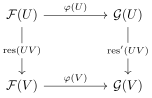An isomorphism is a morphism with a two-sided inverse.

Note that if we regard a sheaf as a contravariant functor, a morphism is then just a natural transformation.

A morphism $$\phi: {\mathcal{F}}\to {\mathcal{G}}$$ defines a morphisms on stalks $$\phi_p: {\mathcal{F}}_p \to {\mathcal{G}}_p$$.

Let $$X \mathrel{\vcenter{:}}={\mathbb{C}}^{\times}$$ with the classical topology, making it into a real manifold, and take $$C^0({-}; {\mathbb{C}}) \in {\mathsf{Sh}}(X, {\mathsf{Ab}})$$ be the sheaf of continuous functions and let $$C^0({-}; {\mathbb{C}})^{\times}$$ the sheaf of of nowhere zero continuous continuous functions. Note that this is a sheaf of abelian groups since the operations are defined pointwise. There is then a morphism \begin{align*} \exp({-}): C^0({-}; {\mathbb{C}}) &\to C^0({-}; {\mathbb{C}})^{\times}\\ f &\mapsto e^f && \text{ on open sets } U\subseteq X .\end{align*}

Since exponentiating and restricting are operations done pointwise, the required square commutes, yielding a morphism of sheaves.

Let $$\phi: {\mathcal{F}}\to {\mathcal{G}}$$ be morphisms of presheaves, then define the presheaves \begin{align*} \ker(\phi)(U) &\mathrel{\vcenter{:}}=\ker(\phi(U)) \\ \operatorname{coker}^{{\mathsf{pre}}}(\phi)(U) &\mathrel{\vcenter{:}}={\mathcal{G}}(U) / \phi({\mathcal{F}}(U))\\ \operatorname{im}(\phi)(U) &\mathrel{\vcenter{:}}=\operatorname{im}(\phi(U)) \\ .\end{align*}

If $${\mathcal{F}}, {\mathcal{G}}\in {\mathsf{Sh}}(X)$$, then for a morphism $$\phi: {\mathcal{F}}\to {\mathcal{G}}$$, the image and cokernel presheaves need not be sheaves!

Consider $$\ker \exp$$ where $$\exp: C^0({-}; {\mathbb{C}})\to C^0({-}; {\mathbb{C}})^{\times}\in {\mathsf{Sh}}({\mathbb{C}}^{\times})$$. One can check that $$\ker \exp = 2\pi i \underline{{\mathbb{Z}}}(U)$$, and so the kernel is actually a sheaf.

We also have $$\operatorname{coker}^{{\mathsf{pre}}} \exp(U) \mathrel{\vcenter{:}}= C^0(U; {\mathbb{C}})/ \exp(C^0(U;{\mathbb{C}})^{\times})$$. On opens, $$\operatorname{coker}^{{\mathsf{pre}}} \exp(U) = \left\{{1}\right\} \iff$$ every nonvanishing continuous function $$g$$ on $$U$$ has a continuous logarithm, i.e. $$g = e^f$$ for some $$f$$. Examples of opens with this property include any contractible (or even just simply connected) open set in $${\mathbb{C}}^{\times}$$. Consider $$U\mathrel{\vcenter{:}}={\mathbb{C}}^{\times}$$ and $$z\in C^0({\mathbb{C}}^{\times}; {\mathbb{C}})^{\times}$$, which is a nonvanishing function. Then the equivalence class $$[z] \in \operatorname{coker}^{{\mathsf{pre}}} \exp({\mathbb{C}}^{\times})$$ is nontrivial, since $$z\neq e^f$$ for any $$f\in C^0({\mathbb{C}}^{\times}; {\mathbb{C}})$$, since any attempted definition of $$\log(z)$$ will have monodromy.

on the other hand we can cover $${\mathbb{C}}^{\times}$$ by contractible opens $$\left\{{U_i}\right\}_{i\in I}$$ where $${ \left.{{[z]}} \right|_{{U_i}} } = 1 \in \operatorname{coker}^{{\mathsf{pre}}} \exp (U_i)$$ and similarly $${ \left.{{1}} \right|_{{\operatorname{id}}} } = 1 \in \operatorname{coker}^{{\mathsf{pre}}} \exp(U_i)$$, showing that the cokernel fails the unique gluing axiom and is not a sheaf.

Given any $${\mathcal{F}}\in \underset{ \mathsf{pre} } {\mathsf{Sh} }(X)$$ there exists an $${\mathcal{F}}^+ \in {\mathsf{Sh}}(X)$$ and a morphism of presheaves $$\theta: {\mathcal{F}}\to {\mathcal{F}}^+$$ such that for any $${\mathcal{G}}\in {\mathsf{Sh}}(X)$$ with a morphism $$\phi: {\mathcal{F}}\to {\mathcal{G}}$$ there exists a unique $$\psi: {\mathcal{F}}^+ \to {\mathcal{G}}$$ making the following diagram commute:The sheaf $${\mathcal{F}}^+ \in {\mathsf{Sh}}(X)$$ is called the sheafification of $${\mathcal{F}}$$. This is an example of an adjunction of functors: \begin{align*} \mathop{\mathrm{Hom}}_{ \underset{ \mathsf{pre} } {\mathsf{Sh} }(X)}({\mathcal{F}}, {\mathcal{G}}^{\mathsf{pre}}) \cong \mathop{\mathrm{Hom}}_{{\mathsf{Sh}}(X)}({\mathcal{F}}^+, {\mathcal{G}}) ,\end{align*} where we use the forgetful functor $${\mathcal{G}}\to {\mathcal{G}}^{\mathsf{pre}}$$. This yields an adjoint pair \begin{align*} \adjunction{a}{b}{c}{d} .\end{align*}

We construct it directly as $${\mathcal{F}}^+ \mathrel{\vcenter{:}}=\left\{{s:U \to {\textstyle\coprod}_{p\in U} {\mathcal{F}}_p }\right\}$$ such that

1. $$s(p) \in {\mathcal{F}}_p$$,
2. The germs are compatible locally, so for all $$p\in U$$ there is a $$V\supseteq p$$ such that for some $$t\in {\mathcal{F}}(V)$$, $$s(p) = t_p$$ for all $$p$$ in $$V$$.

Collections of germs that are locally compatible.

So about any point, there should be an actual function specializing to all germs in an open set.

The point will be that $$\operatorname{coker}\exp$$ will be zero as a sheaf, since it’ll be zero on a sufficiently small set.

# 4 Wednesday, August 25

Recall the definition of sheafification: let $${\mathcal{F}}\in \underset{ \mathsf{pre} } {\mathsf{Sh} }(X; {\mathsf{Ab}}{\mathsf{Grp}})$$. Construct a sheaf $${\mathcal{F}}^+\in {\mathsf{Sh}}(X, {\mathsf{Ab}}{\mathsf{Grp}})$$ and a morphism $$\theta: {\mathcal{F}}\to {\mathcal{F}}^+$$ of presheaves satisfying the appropriate universal property:So any presheaf morphism to a sheaf factors through the sheafification uniquely (via $$\theta$$). Note that this is a instance of a general free/forgetful adjunction.

We can construct it as \begin{align*} {\mathcal{F}}^+(U) \mathrel{\vcenter{:}}=\left\{{s:U\to {\textstyle\coprod}_{p\in U} {\mathcal{F}}_p,\quad s(p) \in {\mathcal{F}}_p, \cdots}\right\} .\end{align*} where the addition condition is that for all $$q\in U$$ there exists a $$V\nu q$$ and $$t\in {\mathcal{F}}(V)$$ such that $$t_p = s(p)$$ for all $$p\in V$$. Note that $$\theta$$ is defined by $$\theta(U)(s) = \left\{{s:p\to s_p}\right\}$$, the function assigning points to germs with respect to the section $$s$$. Idea: this is like replacing an analytic function on an interval with the function sending a point $$p$$ to its power series expansion at $$p$$.

Recall $$\exp: C^0 \to (C^0)^{\times}$$ on $${\mathbb{C}}^{\times}$$, then $$\operatorname{coker}^{\mathsf{pre}}(\exp)(U) = \left\{{1}\right\}$$ on contractible $$U$$, using that one can choose a logarithm on such a set. However $$\operatorname{coker}^{\mathsf{pre}}(\exp)({\mathbb{C}}^{\times}) \neq \left\{{1}\right\}$$ since $$[z]\in (C^0)^{\times}({\mathbb{C}}^{\times})/\exp(C^0({\mathbb{C}}^{\times}))$$.

Letting $$\phi: {\mathcal{F}}\to {\mathcal{G}}$$ be a morphisms of sheaves, then we defined $$\operatorname{coker}(\phi) \mathrel{\vcenter{:}}=(\operatorname{coker}^{\mathsf{pre}}(\phi))^+$$ and $$\operatorname{im}(\phi) \mathrel{\vcenter{:}}=(\operatorname{im}^{\mathsf{pre}}(\phi))^+$$. Then \begin{align*} \operatorname{coker}^{\mathsf{pre}}(\exp) &\to \operatorname{coker}(\exp) \\ s\in {\mathcal{F}}(U) &\mapsto s(p) = s_p .\end{align*} The claim is that $$[z]_p = 1$$ for all $$p\in {\mathbb{C}}^{\times}$$, since we can replace $$[([z], {\mathbb{C}}^{\times})]$$ with $$([z]_U, U)$$ for $$U$$ contractible.

A useful example to think about: $$X = \left\{{p, q}\right\}$$ with

• $${\mathcal{F}}(p) = A$$
• $${\mathcal{F}}(q) = B$$
• $${\mathcal{F}}(X) = 0$$

Then local sections don’t glue to a global section, so this isn’t a sheaf, but it is a presheaf. The sheafification satisfies $${\mathcal{F}}^+(X) = A\times B$$.

## 4.1 Subsheaves

$${\mathcal{F}}'$$ is a subsheaf of $${\mathcal{F}}$$ if

• $${\mathcal{F}}'(U) \leq {\mathcal{F}}(U)$$ for all $$U$$,
• $$\mathop{\mathrm{Res}}'(U, V) = { \left.{{ \mathop{\mathrm{Res}}(U, V) }} \right|_{{{\mathcal{F}}'(U)}} }$$.

$$\phi: {\mathcal{F}}\to {\mathcal{G}}$$ is injective iff $$\ker \phi = 0$$, surjective if $$\operatorname{im}(\phi) = {\mathcal{G}}$$ or $$\operatorname{coker}\phi = 0$$.

Check that $$\ker \phi$$ already satisfies the sheaf property.

Let $$\cdots \to {\mathcal{F}}^{i-1} \xrightarrow{\phi^{i-1}} {\mathcal{F}}^i \xrightarrow{\phi^i} {\mathcal{F}}^{i+1}\to \cdots$$ be a sequence of morphisms in $${\mathsf{Sh}}(X)$$, this is exact iff $$\ker \phi^i = \operatorname{im}\phi^{i-1}$$.

$$\ker \phi$$ is a sheaf.

By definition, $$\ker(\phi)(U) \mathrel{\vcenter{:}}=\ker \qty{ \phi(U): {\mathcal{F}}(U) \to {\mathcal{G}}(U) }$$, satisfying part (a) in the definition of presheaves. We can define restrictions $${ \left.{{\mathop{\mathrm{Res}}(U, V)}} \right|_{{\ker(\phi)(U)}} } \subseteq \ker(\phi)(V)$$. Use the commutative diagram for the morphism $$\phi: {\mathcal{F}}\to {\mathcal{G}}$$.

Now checking gluing: Let $$s_i \in \ker(\phi)(U_i)$$ such that $$\mathop{\mathrm{Res}}(s_i, U_i \cap U_j) = \mathop{\mathrm{Res}}(s_j, U_i \cap U_j)$$ for all $$i, j$$. This holds by viewing $$s_i \in {\mathcal{F}}(U_i)$$, so $$\exists ! s\in {\mathcal{F}}(\displaystyle\bigcup_i U_i)$$ such that $$\mathop{\mathrm{Res}}(s, U_i) = s_i$$. We want to show $$s\in \ker(\phi)\qty{\displaystyle\bigcup U_i}$$, so consider \begin{align*} t\mathrel{\vcenter{:}}=\phi\qty{ \displaystyle\bigcup_i U_i}(s) \in {\mathcal{G}}\qty{\displaystyle\bigcup U_i} ,\end{align*} which is zero. Now \begin{align*} \mathop{\mathrm{Res}}(t, U_i) = \phi(U_i)(\mathop{\mathrm{Res}}(s, U_i)) = \phi(U_i)(s_i) = 0 \end{align*} by assumption, using the commutative diagram. By unique gluing for $${\mathcal{G}}$$, we have $$t=0$$, since $$0$$ is also a section restricting to $$0$$ everywhere.

For $${\mathcal{F}}' \leq {\mathcal{F}}$$ a subsheaf, define the quotient $${\mathcal{F}}/{\mathcal{F}}' \mathrel{\vcenter{:}}=(({\mathcal{F}}/{\mathcal{F}}')^{\mathsf{pre}})^+$$ where \begin{align*} ({\mathcal{F}}/{\mathcal{F}}')^{\mathsf{pre}}(U) \mathrel{\vcenter{:}}={\mathcal{F}}(U)/ {\mathcal{F}}'(U) .\end{align*}

# 5 Friday, August 27

Let $$\phi:{\mathcal{F}}\to{\mathcal{G}}$$ be a morphism in $${\mathsf{Sh}}(X)$$, then $$\phi$$ is an isomorphism iff $$\phi_p: {\mathcal{F}}_p \to{\mathcal{G}}_p$$ is an isomorphism for all $$p\in X$$.

Suppose $$\phi$$ is an isomorphism, so there exists a $$\psi: {\mathcal{G}}\to {\mathcal{F}}$$ which is a two-sided inverse for $$\phi$$. Then $$\psi_p$$ is a two-sided inverse to $$\phi_p$$, making it an isomorphism. This follows directly from the formula: \begin{align*} \phi_p: {\mathcal{F}}_p &\to {\mathcal{G}}_p \\ (s, U) & \mapsto (\phi(U)(s), U) .\end{align*}

It suffices to show $$\phi(U): {\mathcal{F}}(U) \to {\mathcal{G}}(U)$$ is an isomorphism for all $$U$$. This is because we could define $$\psi(U):{\mathcal{G}}(U) \to {\mathcal{F}}(U)$$ and set $$\phi^{-1}(U) \mathrel{\vcenter{:}}=\psi(U)$$, then reversing the arrows in the diagram for a sheaf morphism again yields a commutative diagram.

$$\phi(U)$$ is injective.

For $$s\in {\mathcal{F}}(U)$$, we want to show $$\phi(U)(s) = 0$$ implies $$s=0$$. Consider the germs $$(s, U) \in {\mathcal{F}}_p$$ for $$p\in U$$, we have $$\phi_p(s, U) = (0, U) = 0\in {\mathcal{F}}_p$$. So $$S_p = 0$$ for all $$p\in U$$. Since we have a germ, there exists $$V_p \ni p$$ open such that $${ \left.{{s}} \right|_{{V_p}} } = 0$$. Noting that $$\left\{{V_p {~\mathrel{\Big\vert}~}p\in U}\right\}\rightrightarrows U$$, by unique gluing we get an $$s$$ where $${ \left.{{s}} \right|_{{V_p}} } = 0$$ for all $$V_p$$, so $$s\equiv 0$$ on $$U$$.

$$\phi(U)$$ is surjective.

Let $$t\in {\mathcal{G}}(U)$$, and consider germs $$t_p\in {\mathcal{G}}_p$$. There exists a unique $$s_p\in {\mathcal{F}}_p$$ with $$\phi_p(s_p) = t_p$$, since $$\phi_p$$ is an isomorphism of stalks by assumption. Use that $$s_p$$ is a germ to get an equivalence class $$(s_p, V)$$ where $$V \subseteq U$$. We have $$\phi(V)(s(p), V) \sim (t, U)$$, noting that $$s$$ depends on $$p$$. Having equivalent germs means there exists a $$W(p) \subseteq V$$ with $$p\in W$$ with $$\phi(W(p)) \qty{{ \left.{{s(p)}} \right|_{{W}} }} = { \left.{{t}} \right|_{{W(p)}} }$$. We want to glue these $$\left\{{ { \left.{{s(p)}} \right|_{{W(p)}} } {~\mathrel{\Big\vert}~}p\in U }\right\}$$ together. It suffices to show they agree on intersections. Taking $$p, q\in U$$, both $${ \left.{{s(p)}} \right|_{{W(p) \cap W(q)}} }$$ and $${ \left.{{s(q)}} \right|_{{W(p) \cap W(q)}} }$$ map to $${ \left.{{t}} \right|_{{W(p) \cap W(q)}} }$$ under $$\phi(W(p) \cap W(q) )$$. Injectivity will force these to be equal, so $$\exists ! s \in {\mathcal{F}}(U)$$ with $${ \left.{{s}} \right|_{{W(p)}} } = s(p)$$. We want to now show that $$\phi(U)(s) = t$$. Using commutativity of the square, we have $$\phi(U)(s) { \left.{{}} \right|_{{W(p)}} } = \phi(W(p)) \qty{{ \left.{{s}} \right|_{{W(p)}} } }$$. This equals $$\phi(W(p))(s(p)) = { \left.{{t}} \right|_{{W(p)}} }$$. Therefore $$\phi(U)(s)$$ and $$t$$ restrict to sections $$\left\{{w(p) {~\mathrel{\Big\vert}~}p\in U}\right\}$$. Using unique gluing for $${\mathcal{G}}$$ we get $$\phi(U)(s) = t$$.

Note: we only needed to check overlaps because of exactness of the following sequence: \begin{align*} 0 \to{\mathcal{F}}(U) \to \prod_{i\in I} {\mathcal{F}}(U_i) \to \prod_{i

Let $$f\in {\mathsf{Top}}(X, Y)$$, let $${\mathcal{F}}\in {\mathsf{Sh}}(X)$$ and define the pushforward sheaf $$f_* {\mathcal{F}}\in {\mathsf{Sh}}(Y)$$ by \begin{align*} f_*{\mathcal{F}}(V) \mathrel{\vcenter{:}}={\mathcal{F}}( f^{-1}(V)) .\end{align*}

The inverse image sheaf is define as \begin{align*} (f^{-1}{\mathcal{F}})(U) = \lim_{V\ni f(U) \text{ open}} F(V) .\end{align*}

The inverse image sheaf generalizes stalks, recovering $${\mathcal{F}}_p$$ when $$f(U) = p$$. Note that $$f(U)$$ need not be open, unless $$f$$ is an open map. In this case $$f^{-1}{\mathcal{F}}(f(U))$$.

$$f^*$$ is not the pullback!

Show that $$f_* {\mathcal{F}}$$ makes sense precisely because $$f$$ is continuous. Check that $$f_* {\mathcal{F}}$$ satisfies the sheaf axioms. Use that the set of opens of the form $$f^{-1}(V)$$ are e.g. closed under intersections, and thus inherit all of the sheaf axioms from $${\mathcal{F}}$$>

# 6 Monday, August 30

Let $$R$$ be a commutative unital ring in which $$0\neq 1$$ unless $$R=0$$. The goal is to define a space $$X$$ such that $$R$$ is the ring of functions on $$X$$, imitating the correspondence between $$X$$ a manifold and $$C^0(X; {\mathbb{R}})$$. Recall that an ideal $${\mathfrak{p}}\in \operatorname{Id}(R)$$ is prime iff $${\mathfrak{p}}\subset A$$ is a proper subset and $$fg\in {\mathfrak{p}}\implies f\in {\mathfrak{p}}$$ or $$g\in {\mathfrak{p}}$$.

For $$A$$ a ring, $$\operatorname{Spec}(A)$$ is the set of prime ideals. Topologize this by setting the closed sets to be of the form $$V(I) = \left\{{ {\mathfrak{p}}\in \operatorname{Spec}(A) {~\mathrel{\Big\vert}~}{\mathfrak{p}}\supseteq I }\right\}$$.

Ideals are “contagious” under multiplication, so prime ideals have reverse contagion! It remains to prove that $$\operatorname{Spec}(A)$$ forms a topological space.

For $$A$$ a field, $$\operatorname{Spec}(A) = \left\{{\left\langle{0}\right\rangle}\right\}$$, since any other nonzero element would be a unit and put 1 in the ideal.

For $$k$$ an algebraically closed field, $$\operatorname{Spec}k[t] = \left\{{ \left\langle{0}\right\rangle, \left\langle{t-a}\right\rangle {~\mathrel{\Big\vert}~}a\in A}\right\}$$. This is a PID, so every ideal is of the form $$I = \left\langle{f}\right\rangle$$, so \begin{align*} V(\left\langle{f}\right\rangle) = \begin{cases} \operatorname{Spec}k[t] & f=0 \\ \left\langle{x-a_1, \cdots, a-a_k}\right\rangle & f = \prod_{i=1}^k (x-a_i) \end{cases} .\end{align*}

Note that this is not the cofinite topology, since $$f=0$$ is a generic point.

# 7 Wednesday, September 01

Let $$k = \mkern 1.5mu\overline{\mkern-1.5muk\mkern-1.5mu}\mkern 1.5mu$$ be algebraically closed, then \begin{align*} \operatorname{Spec}k[x] = \left\{{ \left\langle{x-a}\right\rangle {~\mathrel{\Big\vert}~}a\in k}\right\}\cup\left\langle{0}\right\rangle .\end{align*} Similarly, \begin{align*} \operatorname{Spec}k[x, y] = \left\{{ \left\langle{x-a, y-b}\right\rangle {~\mathrel{\Big\vert}~}a,b\in k}\right\} \cup\left\{{\left\langle{f}\right\rangle {~\mathrel{\Big\vert}~}f \text{ irreducible}}\right\} \cup\left\langle{0}\right\rangle .\end{align*} Note that both have non-closed, generic points.

Consider $$X \mathrel{\vcenter{:}}=\operatorname{Spec}{ {\mathbb{Z}}_p }$$ and $$Y\mathrel{\vcenter{:}}=\operatorname{Spec}{\mathbb{C}} { \left[ {t} \right] }$$, then $$\operatorname{Spec}(X) = \left\{{\left\langle{p}\right\rangle, \left\langle{0}\right\rangle}\right\}$$ and $$\operatorname{Spec}(Y) = \left\{{ \left\langle{t}\right\rangle, \left\langle{0}\right\rangle }\right\}$$. Both are two point spaces, with open points $$\left\langle{0}\right\rangle$$ and closed points $$\left\langle{p}\right\rangle$$ and $$\left\langle{f}\right\rangle$$ respectively. This spaces are homeomorphic, and later we’ll see that we can distinguish them as ringed spaces.

Let $$A\in\mathsf{CRing}$$, then $$\operatorname{Spec}A$$ with the closed sets declared to be those of the form $$V(I) = \left\{{p \in \operatorname{Spec}(A) {~\mathrel{\Big\vert}~}p\supseteq I}\right\}$$.

$$V(IJ) = V(I) \cup V(J)$$, so if a prime ideal $$p$$ contains $$IJ$$ then $$p\supseteq I$$ or $$p\supseteq J$$.

$$\impliedby$$: If $$I \subseteq P$$ or $$J \subseteq P$$, then $$IJ \subseteq I$$ and $$IJ \subseteq J$$, so $$IJ \subset p$$.

$$\implies$$: Suppose $$IJ \subset p$$ but $$J \not\subset p$$, so pick $$j\in J \setminus p$$. Then for all $$i\in I$$, we have $$ij\in IJ \subseteq p$$, forcing $$i\in p$$.

An arbitrary intersection satisfies $$\displaystyle\bigcap_i V(I_i) = V(\sum_i I_i)$$.

$$\implies$$: For $$p\in \operatorname{Spec}(A)$$, we want to show that $$p \supseteq\sum I_i$$ iff $$p \supseteq I_i$$ for all $$i$$, so $$I_i \subseteq \sum I_i \subset P$$.

$$\impliedby$$: Ideals are additive groups, regardless of whether or not they’re prime!

• $$\emptyset$$ is closed, since $$\emptyset = V(A)$$
• $$X$$ is closed, since $$X = V(0)$$ and $$O$$ is contained in every prime ideal.
• Closure under finite unions: by induction, it’s enough to show that $$V(I) \cup V(J)$$ is closed. This follows from the 1st lemma above.
• Closure under arbitrary unions: this follows from the 2nd lemma.

$$V(I) = V(\sqrt I)$$. The proof is simple: prime ideals are radical.

Note that $$\operatorname{Spec}{\mathbb{Z}}= \left\{{ \left\langle{p}\right\rangle, \left\langle{0}\right\rangle {~\mathrel{\Big\vert}~}p \text{ is prime}}\right\}$$. Note that maximal ideals are always closed points, and $$\left\langle{0}\right\rangle$$ is not a closed point. This is homeomorphic to, say $$\operatorname{Spec}\mkern 1.5mu\overline{\mkern-1.5mu{\mathbb{Q}}\mkern-1.5mu}\mkern 1.5mu[t]$$.

Suppose $$p \subseteq A$$ is a prime ideal, then the localization of $$A$$ at $$p$$, $$A \left[ { { {(p^c)}^{-1}} } \right]$$ (or $$A_p$$) is defined as \begin{align*} A \left[ { \scriptstyle { {(p^c)}^{-1}} } \right] \mathrel{\vcenter{:}}=\left\{{ a/f {~\mathrel{\Big\vert}~}f\not\in p}\right\}_{/ {\sim }} && {a\over f}\sim {b\over g}\iff \exists \, h\in A \text{ s.t. } h(ag-bf)=0 .\end{align*} This makes the elements of $$p^c$$ invertible, and is a local ring with residue field $$\kappa = \operatorname{ff}(A/p)$$ and maximal ideal $$pA_p$$. Ideals of $$A_p$$ biject with ideals of $$A$$ contained in $$p$$.

Idea: $$A_p$$ should look like germs of functions at the point $$p$$. Note that localizing at the ideal $$p$$ is like deleting $${ \operatorname{cl}} _X(V(p))$$, which is also useful. We now want to construct a sheaf $${\mathcal{O}}= {\mathcal{O}}_{\operatorname{Spec}A}$$ which has stalks $$A_p$$. We’ll construct something that’s obviously a sheaf, at the cost of needing to work hard to prove things about it!

For $$U\in \operatorname{Spec}(A)$$ open, so $$U = V(I)^c$$, define the structure sheaf of $$X$$ as the sheaf given \begin{align*} {\mathcal{O}}(U) \mathrel{\vcenter{:}}=\left\{{ s:U \to \displaystyle\coprod{p\in U} A_p {~\mathrel{\Big\vert}~}s(p) \in A_p, \text{ and } s \text{ is locally a fraction}}\right\} .\end{align*} Here locally a fraction means that for all $$p\in U$$ there is an open $$p\in V \subseteq U$$ and elements $$a, f\in A$$ such that

1. $$f\not\in Q$$ for any $$Q\in V$$ and
2. $$s(Q) = a/f$$ for all $$Q \in V$$.

Restriction is defined for $$V \subseteq U$$ as honest function restriction on $${\mathcal{O}}(U) \to {\mathcal{O}}(V)$$.

Note that this is sheafifying the presheaf $$U = D_f \mapsto A_f$$.

Let $$k \in \mathsf{Field}$$, then $$X\mathrel{\vcenter{:}}=\operatorname{Spec}(k) = \left\{{\left\langle{0}\right\rangle}\right\}$$ and $${\mathcal{O}}_X$$ is determined by \begin{align*} {{\Gamma}\qty{X; {\mathcal{O}}_X} } = \left\{{s: \operatorname{Spec}k \to k {~\mathrel{\Big\vert}~}\cdots}\right\} = k ,\end{align*} since the conditions are vacuous here.

Let $$X = \operatorname{Spec}{\mathbb{C}} { \left[ {t} \right] } = \left\{{ \left\langle{0}\right\rangle, \left\langle{1}\right\rangle}\right\}$$ and $${\mathcal{O}}_X(X) = {\mathbb{C}} { \left[ {t} \right] }$$ and $${\mathcal{O}}_X(\left\langle{0}\right\rangle) = {\mathbb{C}} { \left( {t} \right) }$$.

# 8 Friday, September 03

Last time: we defined $$\operatorname{Spec}A$$ as a topological space and $${\mathcal{O}}_{\operatorname{Spec}A}$$, a sheaf of rings on $$\operatorname{Spec}A$$ which evidently satisfied the gluing condition: \begin{align*} {\mathcal{O}}_{\operatorname{Spec}A}(U) \mathrel{\vcenter{:}}=\left\{{s: U\to \displaystyle\coprod_{p\in U} A \left[ { \scriptstyle { {p}^{-1}} } \right] {~\mathrel{\Big\vert}~}s(p) \in A \left[ { \scriptstyle { {p}^{-1}} } \right] \forall p,\,\, s \text{ is locally a fraction}}\right\} .\end{align*}

Set $$X\mathrel{\vcenter{:}}={\mathbb{A}}^1_{/ {k}} \mathrel{\vcenter{:}}=\operatorname{Spec}k[t]$$ for $$k=\mkern 1.5mu\overline{\mkern-1.5muk\mkern-1.5mu}\mkern 1.5mu$$. Take a point $$\left\langle{t}\right\rangle = \left\langle{t-0}\right\rangle \in \operatorname{Spec}k[t]$$ corresponding to 0, then $${\mathcal{O}}_{X}(X\setminus\left\{{{\operatorname{pt}}}\right\}) = k[t, t^{-1}]$$, i.e. functions of the form $$f/t^k$$ for any $$k$$. Generally for $$p = \left\langle{t-a_i}\right\rangle$$ we get $$s_p \in k[t] \left[ { { {\left\{{t-a_i}\right\}}^{-1}} } \right]$$. Note that for $$p = \left\langle{0}\right\rangle$$, we get $$s_p \in k(t)$$.

$$s$$ is determined by $$s_{\left\langle{0}\right\rangle}$$, so there is an injective map \begin{align*} {\mathcal{O}}(k[t]\setminus\left\{{{\operatorname{pt}}}\right\}) &\to k(t) \\ s &\mapsto s_{\left\langle{0}\right\rangle} .\end{align*}

Note that $$\left\langle{0}\right\rangle$$ is in every open set, so locally near $$p$$ there exists a $$P\in V$$ and $$a,f$$ with $$f\not\in Q$$ for all $$Q$$ and $$s_Q = a/f$$ for all $$Q\in V$$. Since $$\left\langle{0}\right\rangle \in V$$, we have $$s_{\left\langle{0}\right\rangle} = a/f \in k(t)$$ and $$s_p = a/f\in A_p$$. Since $$A_p \subseteq k(t)$$, we get $$s_p = s_{\left\langle{0}\right\rangle}$$ under this inclusion.

$${\mathcal{O}}(\operatorname{Spec}k[t]\setminus\left\{{{\operatorname{pt}}}\right\}) = k[t, t^{-1}]$$.

We showed that the LHS is a subset of $$k(t)$$, so which subsets can be written as things that are locally fractions on the complement of zero.

$$\supseteq$$: This can clearly be done in $$k[t, t^{-1}]$$ since every element is locally the fraction $$f/t^k$$.

$$\subseteq$$: Suppose $$f/g$$ with $$f,g$$ coprime (this is a PID!) with a pole away from zero, so $$g\in Q$$ for some $$Q\neq \left\langle{0}\right\rangle$$. But then $$f/g$$ isn’t in $$A_Q$$.

Note that $$X \mathrel{\vcenter{:}}=\operatorname{mSpec}k[t] \subseteq X' \mathrel{\vcenter{:}}=\operatorname{Spec}k[t]$$ as the set of closed points, and restricting $${\mathcal{O}}_{X'}$$ to $$X$$ yields the sheaf of regular functions. Having the extra generic point was useful!

Show that the maximal ideals in $$\operatorname{mSpec}A$$ correspond precisely to closed points.

Of a function that is locally but not globally a fraction. Take $$A \mathrel{\vcenter{:}}= k[x,y,z,w]/\left\langle{xy-zw}\right\rangle$$, which is the cone over a smooth quadric surface and $$X\mathrel{\vcenter{:}}=\operatorname{Spec}A$$. Then take $$U = \operatorname{Spec}(A) \setminus V(y, w) = V(y)^c \cap V(w)^c$$ and consider the section \begin{align*} s(p) \mathrel{\vcenter{:}}= \begin{cases} x/w & p\in V(w)^c \\ z/y & p\in V(y)^c. \end{cases} \end{align*}

For $$p\in U$$, it makes sense to consider $$x/w$$ and $$z/y$$. Are they equal? The answer is yes because $$xy-zw = 0$$. Check that this can’t be a global fraction, which is a consequence of this random open set not being the complement of localizing at a prime ideal.

Given $$f\in A$$, the distinguished open $$D(f)$$ corresponding to $$f$$ is defined as \begin{align*} D(f) = V(\left\langle{f}\right\rangle)^c \mathrel{\vcenter{:}}=\left\{{p\in \operatorname{Spec}(A) {~\mathrel{\Big\vert}~}f\in p}\right\}^c = \left\{{p\in \operatorname{Spec}A {~\mathrel{\Big\vert}~}f\not\in p}\right\} ,\end{align*}

i.e. the points of $$\operatorname{Spec}(A)$$ where $$f$$ doesn’t vanish.

The sets $$\left\{{D(f) {~\mathrel{\Big\vert}~}f\in A}\right\}$$ for a basis for the topology on $$\operatorname{Spec}(A)$$. This follows from writing $$V(I)^c = \displaystyle\bigcup_{f\in I} D(f)$$.

Let $$A\in \mathsf{CRing}$$ be unital with $$1\neq 0$$ unless $$A=0$$ and consider $$(\operatorname{Spec}A, {\mathcal{O}})$$. Then

1. For any $$p\in \operatorname{Spec}A$$, the stalk $${\mathcal{O}}_p \cong A \left[ { { {p}^{-1}} } \right]$$.
2. For any $$f\in A$$, $${\mathcal{O}}(D(f)) = A \left[ { { {f}^{-1}} } \right]$$.
3. Taking $$f=1$$, $$\Gamma(\operatorname{Spec}A, {\mathcal{O}}) = A$$.

Note that (b) gives the values of $${\mathcal{O}}$$ on a basis of opens, which determines the sheaf.

Define a map \begin{align*} f_p: {\mathcal{O}}_p &\to A_p \\ (U, s) &\mapsto s(p) .\end{align*} This is well-defined since $$p\in W$$ for any $$W \subseteq U \cap V$$ for equivalent germs $$(U, s) \sim (V, t)$$.

Surjectivity: given $$x=a/g \in A_p$$, we want $$(U, s)\in {\mathcal{O}}_p$$ such that $$f_p(U, s) = a/g$$, so just take $$U = D(g)$$ and $$s=a/g$$ (which makes sense!) and evidently maps to $$a/g$$.

Injectivity: supposing $$f_p(U, s) = 0$$ in $$A_p$$, we want $$(U, s) = 0$$. If $$s(p) = 0$$, then there exists some $$h\in P$$ with $$h\cdot s(p) = 0$$. Since $$s(p)$$ is locally a fraction, we can find $$p\in V \subseteq U$$ with $$s=a/g$$ on $$V$$ with $$g\neq 0$$ on $$V$$, so consider $$V \cap D(h)$$. The claim is that $$s$$ is 0 here, which follows from $$h\cdot (a/g) = 0$$.

# 9 Wednesday, September 08

Recall that we defined a first version of affine schemes, namely pairs $$(\operatorname{Spec}A, {\mathcal{O}}_A)$$ where for $$U \subseteq \operatorname{Spec}A$$ open we have $$s\in {\mathcal{O}}(U)$$ locally represented by $${ \left.{{s}} \right|_{{V}} } = a/f$$ for $$V \subseteq U$$ where $$a, f\in A$$ and $$V(f) \cap V = \emptyset$$, so $$f$$ doesn’t vanish on $$V$$. Note that the $$D(f)$$ form a topological basis for $$\operatorname{Spec}A$$, and the gluing condition is difficult, i.e. $${\mathcal{O}}(U)$$ may be hard to compute. We proved that $$OO_{{\mathfrak{p}}} = A \left[ { { {{\mathfrak{p}}}^{-1}} } \right]$$ last time, and today we’re showing

• $${\mathcal{O}}(D(f)) = A \left[ { { {f}^{-1}} } \right]$$,
• $${{\Gamma}\qty{(} }\operatorname{Spec}A, {\mathcal{O}}) \cong A$$.

$$b\implies c$$: Take $$f=1\in A$$, then $${\mathcal{O}}(\operatorname{Spec}A) = {\mathcal{O}}(D(1)) = A$$ using (b), so the only hard part is showing (b).

To prove (b), by definition of $${\mathcal{O}}$$ there is a ring morphism \begin{align*} \psi: A \left[ { \scriptstyle { {f}^{-1}} } \right] &\to {\mathcal{O}}(D(f)) \\ {a\over f^n} &\mapsto {a\over f^n} .\end{align*}

Note that this is just a careful statement, since the morphisms on stalks $$\psi_{{\mathfrak{p}}}: A \left[ { { {f}^{-1}} } \right] \to A \left[ { { {{\mathfrak{p}}}^{-1}} } \right]$$ by not be injective in general.

$$\psi$$ is bijective.

Suppose $$\psi(s) = 0$$, we then want to show $$s=0$$. Write $$s = a/f^n$$, then for all $${\mathfrak{p}}\in D(f)$$ we know $$a/f^n = 0 \in A \left[ { { {{\mathfrak{p}}}^{-1}} } \right]$$. So for each $${\mathfrak{p}}$$ there is some $$h_{{\mathfrak{p}}} \not\in{\mathfrak{p}}$$ where \begin{align*} h_{{\mathfrak{p}}}(a\cdot 1 - f^n\cdot 0) = 0 && \text{in } A \end{align*} in $$A$$. Consider the ideal $${\mathfrak{a}}\mathrel{\vcenter{:}}=\operatorname{Ann}(a) \mathrel{\vcenter{:}}=\left\{{b\in A {~\mathrel{\Big\vert}~}ab=0 \in A}\right\} \ni h_{{\mathfrak{p}}}$$. So take the closed subset $$V({\mathfrak{a}})$$, which does not contain $${\mathfrak{p}}$$ since $${\mathfrak{a}}\not\subseteq{\mathfrak{p}}$$. Now iterating over all $${\mathfrak{p}}\in D(f)$$, we get $$V({\mathfrak{a}}) \cap D(f) = \emptyset$$. So $$V({\mathfrak{a}}) \subseteq V(f) = D(f)^c$$, thus $$f\in \sqrt{{\mathfrak{a}}}$$ and $$f^m a = 0$$ for some $$m$$. Then $$f^m(a\cdot 1 - f^n\cdot 0) = 0$$ in $$A$$, so $$a/f^n = 0$$ in $$A \left[ { { {f}^{-1}} } \right]$$.

Step 1: Expressing $$s\in {\mathcal{O}}(D(f))$$ nicely locally.

By definition of $${\mathcal{O}}_{D(f)}$$, there exist $$V_i$$ with $${ \left.{{s}} \right|_{{V_i}} } = a_i/g_i$$ for $$a_i, g_i\in A$$. We’d like $$g_i = h_i^{m_i}$$ for some $$m_i$$, so $$g$$ is a power of $$h_i$$, but this may not be true a priori. Fix $$V_i = D(h_i)$$, then $$a_i / g_i\in {\mathcal{O}}(D(h_i))$$ implies that $$g_i\not\in {\mathfrak{p}}$$ for any $${\mathfrak{p}}\in D(h_i)$$. This implies that $$D(h_i) \subseteq D(g_i)$$, and taking complements yields $$V(h_i) \supseteq V(g_i)$$, and $$h_i \in \sqrt{\left\langle{g_i}\right\rangle}$$ and $$h_i^{n} = g_i$$. Writing $$g_i = h_i^n/c$$ we have $$a_i/g_i = ca_i / h_i^n$$. Note that $$D(h_i) = D(h_i^n)$$. Now replace $$a_i$$ with $$ca_i$$ and $$g_i$$ with $$h_i$$ to get \begin{align*} { \left.{{s}} \right|_{{D(h_i)}} } = a_i / h_i .\end{align*}

Step 2: Quasicompactness of $$D(f)$$.

Note that $$\left\{{D(h_i)}\right\}_{i\in I} \rightrightarrows D(f)$$, so take a finite subcover $$\left\{{D(h_i)}\right\}_{i\leq m}$$.

Proof of quasicompactness: since $$D(f) \supseteq\displaystyle\bigcup_{i\in I} D(h_i)$$, we get \begin{align*} V(f) \subseteq \displaystyle\bigcap_{i\in I} V(h_i) = V\qty{ \sum h_i} .\end{align*} So $$f^u \in \sum h_i$$, and up to reordering we can conclude $$f^u = \sum_{i\leq m} b_i h_i$$ for some $$b_i \in A$$. Then $$D(f) \subseteq \displaystyle\bigcup_{i\leq m} D(h_i)$$.

Since we can write $$\operatorname{Spec}A = D(1)$$, it is quasicompact.

Step 3: Showing surjectivity.

Next time.

# 10 Friday, September 10

## 10.1 Sections and Stalks of $${\mathcal{O}}_{\operatorname{Spec}A}$$ as Localizations

Last time: any affine scheme is quasicompact, so for each ring $$A$$ and an open cover $${\mathcal{U}}\rightrightarrows D(f)$$ then there is a finite subcover $$\left\{{D(h_i)}\right\}\rightrightarrows D(f)$$. We were looking at proposition: for the ringed space $$(\operatorname{Spec}A, {\mathcal{O}}_A)$$,

• $${\mathcal{O}}_{\mathfrak{p}}\cong A \left[ { { {{\mathfrak{p}}}^{-1}} } \right]$$ for all $${\mathfrak{p}}\in \operatorname{Spec}A$$,
• $${\mathcal{O}}(d(f))\cong A \left[ { { {f}^{-1}} } \right]$$ for all $$f\in A$$,
• $${{\Gamma}\qty{\operatorname{Spec}A; {\mathcal{O}}_A} } \cong A$$.

Note that $${\mathcal{O}}_A$$ is uniquely characterized by these properties.

We can write $$D(1) = \operatorname{Spec}A$$ and write $$\left\{{ D(h_i) }\right\} \rightrightarrows\operatorname{Spec}A$$ to obtain $$1^n = \sum b_i h_i$$. This is analogous to a partition of unity, where $$b_i h_i$$ vanishes on $$D(h_i)^c = V(h_i)$$

Let $$\psi:A \left[ { { {f}^{-1}} } \right] \hookrightarrow{\mathcal{O}}(D(f))$$ where we just take restrictions of functions. We know this is injective, and we want to show surjectivity.

Step 1: Let $$s\in {\mathcal{O}}(D(f))$$. For each open $$D(h_i)$$, write $${ \left.{{s}} \right|_{{D(h_i)}} } = a_i /h_i$$ for some $$a_i \in A$$.

Step 2: By quasicompactness, write $$f^n = \sum_{1 \leq i\leq m} b_i h_i$$.

Step 3: Glue the $$a_i/h_i$$ into an element $$a/f$$ of $$A \left[ { { {f}^{-1}} } \right]$$.

Part 1: For any $$1\leq i\neq j\leq m$$, $$D(h_i h_j) = D(h_i) \cap D(h_j)$$. Note that $$a_i/h_i = a_j/h_j$$ in $${\mathcal{O}}(D(h_i h_j))$$, and these are elements of $$A \left[ { { {h_i h_j}^{-1}} } \right]$$ since $$a_i /h_i = a_ih_j/h_i h_j$$. Using injectivity of $$\psi$$ for $$h_i h_j$$, we get an equality $$a_i/h_i = a_j/h_j$$ in $$A_{h_i h_j}$$. Then for $$\ell$$ large enough, $$(h_i h_j)^\ell( a_i h_j - a_j h_i) = 0 \in A$$. Regrouping yields $$h_j^{n+1}(h_i^n a_i) - h_i^{n+1}(h_j a_j) = 0$$, so \begin{align*} {a_i h_i^n \over h_i ^{n+1}} = {a_j h_j^r \over h_j^{n+1}} \mathrel{\vcenter{:}}={\tilde a_i \over \tilde h_i} = {\tilde a_j \over \tilde h_j} ,\end{align*} noting that $$D(\tilde h_i) = D(\tilde h_i)$$ since $$\tilde h_i$$ is a power of $$h_i$$.

Now use POU gluing to write $$f^n = \sum_i \tilde b_i \tilde h_i$$ and $$a \mathrel{\vcenter{:}}=\sum \tilde a_i \tilde h_i\in A$$ be a global function on $$D(f)$$. Then (claim) $$a_j/f^n = \tilde a_j/\tilde h_j$$ on $$D(\tilde h_j)$$. We can rewrite \begin{align*} \tilde h_j a = \sum_i \tilde b_i \tilde a_i \tilde h_j = \sum_i \tilde b_i \tilde a_j \tilde h_i .\end{align*} But then $$a/f^n = { \left.{{s}} \right|_{{D(\tilde h_i)}} }$$, so $$s= a/f^n$$.

Consider $${\mathbb{P}}^1_{/ {k}}$$ as a scheme – we know the space, and the claim is that we can glue sheaves of affines to obtain a structure sheaf for it. Cover $${\mathbb{P}}^1$$ by $$U_0 = {\mathbb{P}}^1\setminus\left\{{\infty}\right\} \cong {\mathbb{A}}^1$$ and $$U_1 = {\mathbb{P}}^1\setminus\left\{{0}\right\} \cong {\mathbb{A}}^1$$. The gluing data is the following:Here the transition maps are \begin{align*} \phi_1 \circ \phi_0^{-1}: \phi_0( U_0 \cap U_1) &\to \phi_1(U_0 \cap U_1) \\ t &\mapsto t^{-1} .\end{align*} What is the map on sheaves? We need a map $${ \left.{{{\mathcal{O}}}} \right|_{{U_0\setminus\left\{{0}\right\}}} } { { \, \xrightarrow{\sim}\, }}{ \left.{{{\mathcal{O}}}} \right|_{{U_1 \setminus\left\{{{\operatorname{pt}}}\right\}}} }$$.

A ringed space $$(X, {\mathcal{O}}_X) \in {\mathsf{Top}}\times {\mathsf{Sh}}(X, \mathsf{Ring})$$ as a topological space with a sheaf of rings. A morphism is a pair $$(f, f^\#) \in C^0(X, Y) \times \in \mathop{\mathrm{Mor}}_{\mathsf{Sh}}({\mathcal{O}}_Y, f_* {\mathcal{O}}_X)$$.

The scheme $$(\operatorname{Spec}A, {\mathcal{O}}_{\operatorname{Spec}A})$$ is a ringed space.

Consider $${\mathbb{R}}$$ in the Euclidean topology, then $$({\mathbb{R}}, C^0({-}, {\mathbb{R}}))$$ with the sheaf of continuous functions is a ringed space. Then consider the morphism given by projection onto the first coordinate of $${\mathbb{R}}^2$$: \begin{align*} (\pi, \pi^\#): ({\mathbb{R}}^2, C^0({-}, {\mathbb{R}})) &\to ({\mathbb{R}}, C^{\infty }({-}, {\mathbb{R}})) \\ (x, y) &\mapsto x .\end{align*} For $$\pi^\#$$, we can consider $$\pi_* C^0({-}, {\mathbb{R}})(U) \mathrel{\vcenter{:}}= C^0(\pi^{-1}(U)) = C^0(U\times {\mathbb{R}})$$, so \begin{align*} \pi^\#: C^\infty(U, {\mathbb{R}}) &\to C^0(U\times {\mathbb{R}}) \\ f &\mapsto f\circ \pi ,\end{align*} which is a well-defined map of rings since smooth functions are continuous.

Not every scheme is built out of affine opens!

# 11 Monday, September 13

## 11.1 Affine Schemes

Let $$(X, {\mathcal{O}}_X) \in \mathsf{RingSp}$$ and $$U \subseteq X$$ be open, then for $$V \subseteq U$$ open, define the restricted sheaf $${\mathcal{O}}_{X}{ \left.{{}} \right|_{{V}} }(V) \mathrel{\vcenter{:}}={\mathcal{O}}_X(V)$$.

\begin{align*} {\mathsf{Sh}}_{/ {X}} \ni{ \left.{{{\mathcal{O}}_X}} \right|_{{U}} }\neq {\mathcal{O}}_X(U) \in \mathsf{Ring} .\end{align*}

Recall the definition of a ringed space $$(X, {\mathcal{O}}_X)$$. The quintessential example: $$X$$ a smooth manifold and $${\mathcal{O}}_X \mathrel{\vcenter{:}}= C^{\infty}({-}; {\mathbb{R}})$$ the sheaf of smooth functions. For defining morphisms, consider a map $$f:X\to Y$$, then an alternative way of defining $$f$$ to be smooth is that there is a pullback \begin{align*} f^*: C^0(V, {\mathbb{R}}) &\to C^0(U, {\mathbb{R}}) \\ g &\mapsto g \circ f \end{align*} for $$U \subseteq X, V \subseteq Y$$, and that $$f^*$$ in fact restricts to $$f^*: C^\infty(V; {\mathbb{R}}) \to C^\infty(U; {\mathbb{R}})$$, i.e. preserving smooth functions.

A morphism of ringed spaces is a pair \begin{align*} (M, {\mathcal{O}}_M) \xrightarrow{(\varphi, \varphi^\#)} (N, {\mathcal{O}}_N) .\end{align*} where $$\varphi \in C^0(M, N)$$ and $$\varphi^\# \in \mathop{\mathrm{Mor}}_{{\mathsf{Sh}}_{/ {N}} }({\mathcal{O}}_N, \varphi_* {\mathcal{O}}_M)$$.

This is an isomorphism of ringed spaces if

1. $$\varphi$$ is a homeomorphism, and
2. $$\varphi^\#$$ is an isomorphism of sheaves.

In the running example, \begin{align*} \varphi^\#(U): {\mathcal{O}}_N(U) \to \varphi_* {\mathcal{O}}_M(M) = {\mathcal{O}}_M(\varphi^{-1}(U)) .\end{align*} This implies that maps of ringed spaced here induce smooth maps, and so there is an embedding $${\mathsf{sm}}{\mathsf{Mfd}}_{/ {{\mathbb{R}}}} \hookrightarrow\mathsf{RingSp}$$.

We’ll try to set up schemes the same way one sets up smooth manifolds. A topological manifold is a space locally homeomorphic to $${\mathbb{R}}^n$$, and a smooth manifold is one in which it’s locally isomorphic as a ringed space to $$({\mathbb{R}}^n, C^\infty({-}; {\mathbb{R}}))$$ with its sheaf of smooth functions.

A smooth manifold is a ringed space $$(M, {\mathcal{O}}_M)$$ that is locally isomorphic to $$({\mathbb{R}}^d, C^\infty({-}; {\mathbb{R}}))$$, i.e. there is an open cover $${\mathcal{U}}\rightrightarrows M$$ such that \begin{align*} (U_i, { \left.{{{\mathcal{O}}_M}} \right|_{{U_i}} }) \cong ({\mathbb{R}}^n, C^{\infty}({-}; {\mathbb{R}})) .\end{align*}

An example of a morphism of ringed spaces that is not an isomorphism: take $$({\mathbb{R}}, C^0) \to ({\mathbb{R}}, C^\infty)$$ given by $$(\operatorname{id}, \operatorname{id}^\#)$$ where $$\operatorname{id}^\#: C^\infty \to \operatorname{id}_* C^0$$ is given by $$\operatorname{id}^\#(U): C^\infty(U) \to C^0(U)$$ is the inclusion of continuous functions into smooth functions.

We’ll define schemes similarly: build from simpler pieces, namely an open cover with isomorphisms to affine schemes. A major difference is that there may not exist a unique isomorphism type among all of the local charts, i.e. the affine scheme can vary across the cover.

Recall that for $$A$$ a ring we defined $$(\operatorname{Spec}A, {\mathcal{O}}_{\operatorname{Spec}A})$$, where $$\operatorname{Spec}A \mathrel{\vcenter{:}}=\left\{{\text{Prime ideals } {\mathfrak{p}}{~\trianglelefteq~}A}\right\}$$, equipped with the Zariski topology generated by closed sets $$V(I) \mathrel{\vcenter{:}}=\left\{{{\mathfrak{p}}{~\trianglelefteq~}A {~\mathrel{\Big\vert}~}I\supseteq{\mathfrak{p}}}\right\}$$. We then defined \begin{align*} {\mathcal{O}}_{\operatorname{Spec}A}(U) \mathrel{\vcenter{:}}=\left\{{s: U\to \displaystyle\coprod_{{\mathfrak{p}}\in U} A \left[ { \scriptstyle { {{\mathfrak{p}}}^{-1}} } \right] {~\mathrel{\Big\vert}~} s({\mathfrak{p}}) \in A \left[ { \scriptstyle { {{\mathfrak{p}}}^{-1}} } \right], \, s\text{ locally a fraction} }\right\} .\end{align*} We saw that

1. We can identify stalks: $${\mathcal{O}}_{\operatorname{Spec}A, {\mathfrak{p}}} = A \left[ { { {{\mathfrak{p}}}^{-1}} } \right]$$
2. We can identify sections on distinguished opens: \begin{align*} {\mathcal{O}}_{\operatorname{Spec}A}(D_f) = A \left[ { \scriptstyle { {f}^{-1}} } \right] = \left\{{a/f^k {~\mathrel{\Big\vert}~}a\in A, k\in {\mathbb{Z}}_{\geq 0}}\right\} ,\end{align*} where $$D_f \mathrel{\vcenter{:}}= V(f)^c = \left\{{{\mathfrak{p}}\in \operatorname{Spec}A {~\mathrel{\Big\vert}~}f\not\in {\mathfrak{p}}}\right\}$$.

As a corollary, we get $${\mathcal{O}}_{\operatorname{Spec}A}(\operatorname{Spec}A) = A$$, noting $$\operatorname{Spec}A = d_1$$ and $$A \left[ { { {1}^{-1}} } \right] = A$$. Thus we can recover the ring $$A$$ from the ringed space $$(X, {\mathcal{O}}_X) \mathrel{\vcenter{:}}=(\operatorname{Spec}A, {\mathcal{O}}_{\operatorname{Spec}A})$$ by taking global sections, i.e. $${{\Gamma}\qty{\operatorname{Spec}A; {\mathcal{O}}_{\operatorname{Spec}A}} } = A$$.

## 11.2 Affine Varieties

Let $$k = \mkern 1.5mu\overline{\mkern-1.5muk\mkern-1.5mu}\mkern 1.5mu$$ and set $${\mathbb{A}}^n_{/ {k}} = k^n$$ whose regular functions are given by $$k[x_1, \cdots, x_{n}]$$, regarded as maps to $$k$$.

An affine variety is any set of the form \begin{align*} X \mathrel{\vcenter{:}}= V(f_1,\cdots, f_n) = \left\{{p\in {\mathbb{A}}^n_{/ {k}} {~\mathrel{\Big\vert}~}f_1(p) = \cdots = f_m(p) = 0}\right\} \end{align*} for $$f_i \in k[x_1, \cdots, x_{n}]$$,

Writing $$I = \left\langle{f_1,\cdots, f_m}\right\rangle$$, we have $$X = V(\sqrt I)$$. Letting $$I(S) = \left\{{f\in k[x_1, \cdots, x_{n}]{~\mathrel{\Big\vert}~}{ \left.{{f}} \right|_{{S}} } = 0}\right\}$$, then by the Nullstellensatz, $$IV(I) = \sqrt{I}$$. This gives a bijection between affine varieties in $${\mathbb{A}}^n_{/ {k}}$$ and radical ideals $$I {~\trianglelefteq~}k[x_1, \cdots, x_{n}]$$.

The coordinate ring of an affine variety $$X$$ is $$k[X] \mathrel{\vcenter{:}}= k[x_1, \cdots, x_{n}]/I(X)$$, regarded as polynomial functions on $$X$$.

We quotient here because if the difference of functions is in $$I(X)$$, these functions are equal when restricted to $$X$$. For example, $$y=x$$ in $$k[x, y]/ \left\langle{x-y}\right\rangle$$, which are different functions where for $$X\mathrel{\vcenter{:}}=\Delta$$, we have $${ \left.{{x}} \right|_{{\Delta}} } = { \left.{{y}} \right|_{{\Delta}} }$$.

As an application of the Nullstellensatz, there is a correspondence

\begin{align*} \adjunction{I({-})}{V({-})}{\left\{{\text{Points } p\in X}\right\}}{\operatorname{mSpec}k[X]} \end{align*}

Why is an affine variety $$X$$ an example of an affine scheme $$\operatorname{Spec}k[X]$$? These don’t have the same underlying topological space: \begin{align*} \tau(X) &\mathrel{\vcenter{:}}=\left\{{V(I) \mathrel{\vcenter{:}}=\left\{{p\in X {~\mathrel{\Big\vert}~}f_i(p) = 0 \,\, \forall f_i \in I}\right\} {~\mathrel{\Big\vert}~}I{~\trianglelefteq~}k[X]}\right\} \\ \tau(\operatorname{mSpec}k[X]) &\mathrel{\vcenter{:}}=\left\{{ V(I) \mathrel{\vcenter{:}}=\left\{{ {\mathfrak{m}}\in \operatorname{mSpec}k[X] {~\mathrel{\Big\vert}~}{\mathfrak{m}}\supseteq I}\right\} {~\mathrel{\Big\vert}~}I{~\trianglelefteq~}k[X] }\right\} .\end{align*}

However, they are closely related: \begin{align*} { \left.{{\tau(\operatorname{mSpec}k[X])}} \right|_{{\operatorname{Spec}k[X]}} } = \tau(X_{\mathrm{zar}}) ,\end{align*} i.e. the space $$\operatorname{Spec}k[X]$$ with the restricted topology from $$\operatorname{mSpec}k[X]$$ is homeomorphic to $$X$$ with the Zariski topology. I.e. restricting to closed points recovers regular functions on $$X$$.

Defining things that are locally isomorphic to schemes would work for objects but not morphisms!

# 12 Wednesday, September 15

Last time: for $${\mathsf{Aff}}{\mathsf{Var}}$$ we considered $$X = V(I) \subseteq {\mathbb{A}}^n_{/ {k}}$$, and for $${\mathsf{Aff}}{\mathsf{Sch}}$$ we considered $$\operatorname{Spec}k[X]$$ where $$k[X] \mathrel{\vcenter{:}}= k[x_1, \cdots, x_{n}]/ I(X)$$. Both had the Zariski topology, and $$X = \operatorname{mSpec}k[X] \subseteq \operatorname{Spec}k[X]$$. We had structure sheaves $${\mathcal{O}}_{\operatorname{Spec}k[X]}$$, and for $$X$$, we have $$U' \mathrel{\vcenter{:}}= U \cap\operatorname{mSpec}k[X]$$. On $$\operatorname{mSpec}k[X]$$, we have the notion of a regular function, and $${\mathcal{O}}_X(U') = {\mathcal{O}}_{\operatorname{Spec}k[X]}(U')$$. The previous setup only worked for rings finitely generated over a field, whereas for schemes, we can take things like $$\operatorname{Spec}{\mathbb{Z}}$$, so they’re much more versatile (e.g. for number theory).

Compare $${\mathbb{A}}^2_{/ {k}} \in {\mathsf{Aff}}{\mathsf{Var}}$$ to $$\operatorname{Spec}k[x, y]$$. Note that $$\left\langle{0}\right\rangle \in \operatorname{Spec}k[x, y]$$, and taking its closure yields \begin{align*} { \operatorname{cl}} (\left\langle{0}\right\rangle) &= \displaystyle\bigcap_{V(I)\supseteq\left\langle{0}\right\rangle } V(I) \\ &= \displaystyle\bigcap_{V(I)\ni 0 } V(I) \\ &= V(0) \\ &= \operatorname{Spec}k[x, y] ,\end{align*} so $$0$$ is a dense point!But there are prime ideals of height $$>1$$. For example, any irreducible subvariety of $${\mathbb{A}}^2$$ yields a generic point.

Try to draw $$\operatorname{Spec}{\mathbb{Z}}$$ and $$\operatorname{Spec}{\mathbb{Z}}[t]$$.

We’ll now try a naive definition of schemes, which we’ll find won’t quite work.

A scheme is a ringed space $$(X, {\mathcal{O}}_X)$$ which is locally an affine scheme, i.e. there exists an open cover $${\mathcal{U}}\rightrightarrows X$$ such that there is a collection of rings $$A_i$$ with \begin{align*} (U_i, { \left.{{{\mathcal{O}}_{X}}} \right|_{{U_i}} } ) { { \, \xrightarrow{\sim}\, }}(\operatorname{Spec}A_i, {\mathcal{O}}_{\operatorname{Spec}A_i}) .\end{align*}

This produces the right objects, but not the correct morphisms. This is a subtle issue! With this definition, a morphism of schemes would be a morphism of ringed spaces $$(f, f^\#)$$ with $$f\in {\mathsf{Top}}(X, Y)$$ and $$f^\# \in {\mathsf{Sh}}_{/ {Y}} ({\mathcal{O}}_Y, f_* {\mathcal{O}}_X)$$, where $$f^\#$$ is supposed to capture “pullback of functions.” The issue: $$f^\#$$ may not notice that $$p \xrightarrow{f} f(p)$$! In particular, it may not preserve the fact that $$f(p) = 0$$.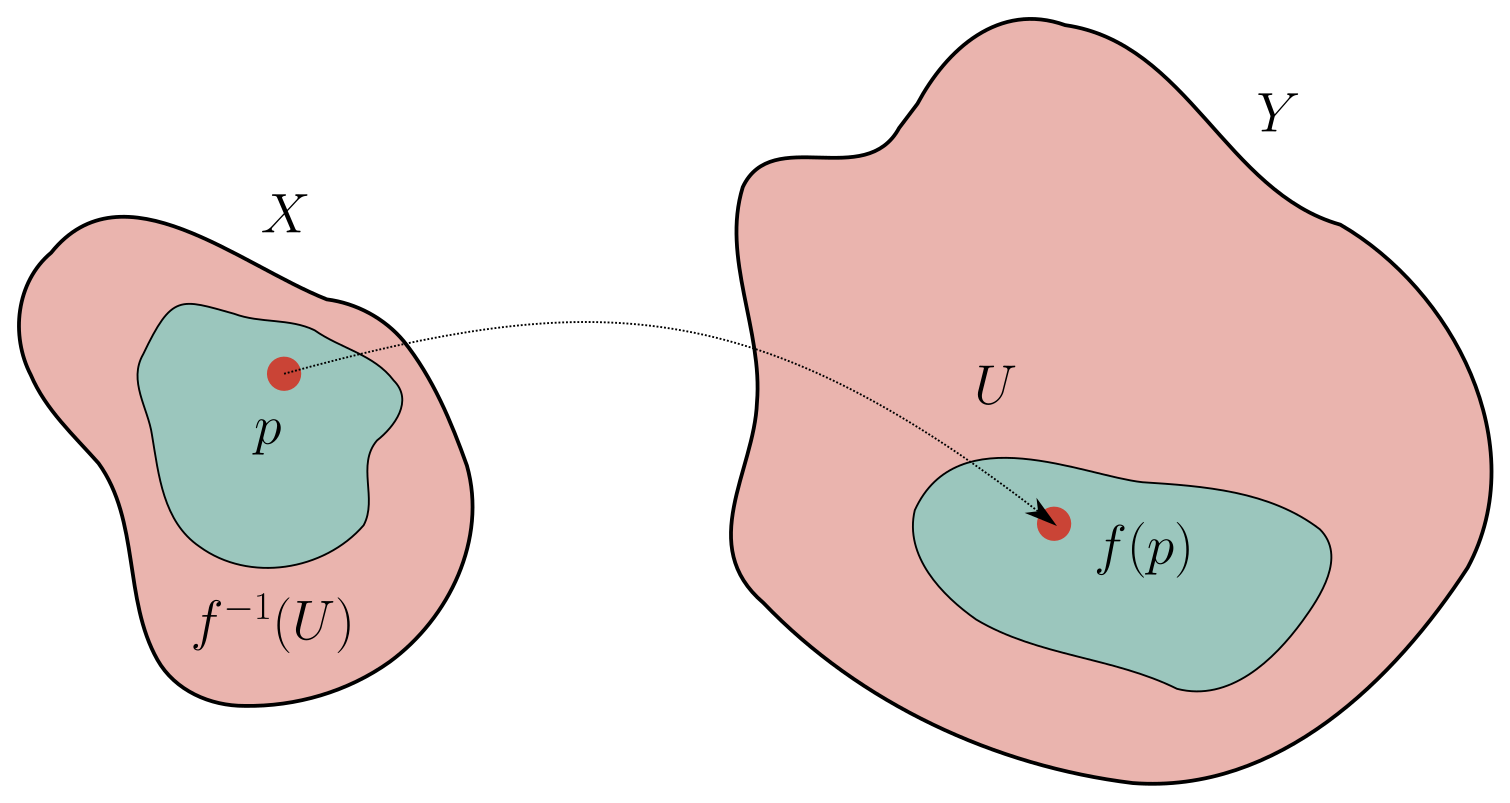Hartshorne exercises for how this issue can actually arise.

Let $$(f, f^\#)$$ be a map of ringed spaces, then there is an induced map \begin{align*} f_p^\#: {\mathcal{O}}_{Y, f(p)} &\to {\mathcal{O}}_{X, p} \\ (U, s) &\mapsto (f^{-1}(U), f^\#(U)(s)) .\end{align*}

A locally ringed space $$(X, {\mathcal{O}}_X)$$ is a ringed space for which the stalks $${\mathcal{O}}_{X, p} \in \mathsf{Loc}\mathsf{Ring}$$ are local rings, i.e. there exists a unique maximal ideal.

For $$(X, {\mathcal{O}}_X) \mathrel{\vcenter{:}}=(\operatorname{Spec}A, {\mathcal{O}}_{\operatorname{Spec}A})$$, we saw that $${\mathcal{O}}_{X, p} = A \left[ { { {p}^{-1}} } \right]$$, which is local.

A morphism of locally ringed spaces is a morphism of ringed spaces \begin{align*} (f, F^\#): (X, {\mathcal{O}}_X) \to (Y, {\mathcal{O}}_Y) \end{align*} such that $$f^\#_p: {\mathcal{O}}_{Y, f(p)} \to {\mathcal{O}}_{X, p}$$ is a homomorphism of local rings, i.e. $$f^\#({\mathfrak{m}}_{f(p)}) \subseteq {\mathfrak{m}}_p$$.

Here we should also require that $$f^\# \neq 0$$.

Morally: this extra condition enforces that pulling back functions vanishing at $$f(p)$$ yields functions which vanish at $$p$$.

Alternatively one could require that $$(f^\#)^{-1}({\mathfrak{m}}_p) = {\mathfrak{m}}_{f(p)}$$, and (claim) this is equivalent to the above definition. Use that $$(f^\#)^{-1}({\mathfrak{m}}_p)$$ is a prime ideal containing $${\mathfrak{m}}_p$$.

Take $$(X, {\mathcal{O}}_X) \mathrel{\vcenter{:}}=({\mathbb{R}}, C^0({-}; {\mathbb{R}}))$$. Why this is in $$\mathsf{Loc}\mathsf{RingSp}$$: write a stalk as \begin{align*} C_p^0 = \left\{{(f, I) {~\mathrel{\Big\vert}~}I\ni p \text{ an interval}, f\in {\mathsf{Top}}(I, {\mathbb{R}})}\right\}_{/ {\sim}} .\end{align*} Why is this local? Take $${\mathfrak{m}}_p \mathrel{\vcenter{:}}=\left\{{(f, I) {~\mathrel{\Big\vert}~}f(p) = 0}\right\}$$, which is maximal since $$C_p^0/{\mathfrak{m}}\cong {\mathbb{R}}$$ is a field. Then $${\mathfrak{m}}_p^c = \left\{{(f, I) {~\mathrel{\Big\vert}~}f(p) \neq 0}\right\}$$, and any continuous function that isn’t zero at $$p$$ is nonzero in some neighborhood $$J \supseteq I$$, so $${ \left.{{f}} \right|_{{J}} }\neq 0$$ anywhere. Then $$(f, I) \sim ({ \left.{{f}} \right|_{{J}} }, J)$$, which is invertible in the ring, so any element in the complement is a unit.

Consider \begin{align*} ({\mathbb{R}}, C^0({-}; {\mathbb{R}})) \xrightarrow{(f, f^\#)} ({\mathbb{R}}, C^\infty({-}; {\mathbb{R}})) .\end{align*} Take $$f = \operatorname{id}$$ and the inclusion \begin{align*} f^\# : C^\infty({-}; {\mathbb{R}})\hookrightarrow\operatorname{id}_* C^0({-}; {\mathbb{R}}) = C^0({-}; {\mathbb{R}}) .\end{align*} Then \begin{align*} f_p^\#: C_p^\infty({-}; {\mathbb{R}}) \to C_p^0({-}; {\mathbb{R}}) .\end{align*} satisfies $$f_p^\#({\mathfrak{m}}^\infty_{\operatorname{id}(p)}) = {\mathfrak{m}}^0_p$$. We also have $$(f_p^\#)^{-1}({\mathfrak{m}}_p^0) = {\mathfrak{m}}_p^\infty$$, since the germs are just equal.

A scheme $$(X, {\mathcal{O}}_X)$$ is a locally ringed space which is locally isomorphic to $$(\operatorname{Spec}A, {\mathcal{O}}_{\operatorname{Spec}A})$$ in $$\mathsf{Loc}\mathsf{RingSp}$$. A morphism of schemes is a morphism in $$\mathsf{Loc}\mathsf{RingSp}$$.

Note that we can restrict to opens, since this doesn’t change the stalks.

As a proof of concept that this is a good notion, we’ll see that there’s a fully faithful contravariant functor $$\operatorname{Spec}: \mathsf{CRing}\to {\mathsf{Sch}}$$, so \begin{align*} \operatorname{Spec}(\mathop{\mathrm{Mor}}_\mathsf{Ring}(B, A)) = \mathop{\mathrm{Mor}}_{\mathsf{Sch}}( \operatorname{Spec}A, \operatorname{Spec}B) .\end{align*}

# 13 Friday, September 17

Recall from last time: a locally ringed space $$(X, {\mathcal{O}}_X)$$ is a ringed space (so $$X\in {\mathsf{Top}}, {\mathcal{O}}_X \in {\mathsf{Sh}}(X, \mathsf{Ring}))$$ such that the stalks $${\mathcal{O}}_{X, p} \in \mathsf{Loc}\mathsf{Ring}$$ for all points $$p\in X$$. Some examples:

• $$(M, {\mathcal{O}}_M)$$ with $$M$$ a manifold and $${\mathcal{O}}_M$$ any sheaf of reasonable functions,
• $$(\operatorname{Spec}A, {\mathcal{O}}_{\operatorname{Spec}A})$$

We defined a scheme as a locally ringed space which is locally isomorphic in $$\mathsf{Loc}\mathsf{RingSp}$$ to $$(\operatorname{Spec}A, {\mathcal{O}}_{\operatorname{Spec}A})$$. Recall that locally meant there exists an open cover $${\mathcal{U}}$$ with the property holding for every element in the cover. Note that most “local” conditions from commutative algebra (that can be checked at all localizations) will correspond to properties that hold on all open covers.

There are generally two ways to define properties of schemes: either it holds for every affine open cover, or there exists an affine open cover.

1. If $$A\in \mathsf{Ring}$$, then $$(\operatorname{Spec}A, {\mathcal{O}}_{\operatorname{Spec}A})\in \mathsf{Loc}\mathsf{RingSp}$$.
2. If $$f\in \mathsf{CRing}(A, B)$$ is a ring morphism, it induces a morphism $$(f, f^\#) \in \mathsf{Loc}\mathsf{RingSp}(\operatorname{Spec}B, \operatorname{Spec}A)$$.
3. Moreover, every $$(f, f^\#) \in \mathsf{Loc}\mathsf{RingSp}(\operatorname{Spec}B, \operatorname{Spec}A)$$ is induced by some $$f\in {\mathsf{Top}}(A, B)$$.

Note that morphisms in $$\mathsf{RingSp}$$ don’t necessarily restrict to morphisms in $$\mathsf{Loc}\mathsf{RingSp}$$, i.e. this is not a full subcategory, since morphisms of rings need not be morphisms of local rings (i.e. those preserving the maximal ideal).

Part (a): This follows from the theorem last week that $${\mathcal{O}}_{\operatorname{Spec}A, p} = A \left[ { { {p}^{-1}} } \right]$$.

Part(b): There’s only ever one thing to do! Define the set-theoretic map \begin{align*} f: \operatorname{Spec}B \to \operatorname{Spec}A \\ p &\mapsto \phi^{-1}(p) .\end{align*} Why this is also continuous: we’ll show preimages preserve closed sets. We can write \begin{align*} f^{-1}(V(I)) &= f^{-1}\qty{\left\{{Q {~\mathrel{\Big\vert}~}Q \supseteq I}\right\}} \\ &= \left\{{{\mathfrak{p}}{~\mathrel{\Big\vert}~}\phi^{-1}({\mathfrak{p}}) \supseteq I}\right\} \\ &= \left\{{{\mathfrak{p}}{~\mathrel{\Big\vert}~}{\mathfrak{p}}\supseteq I}\right\} \\ &= V(\left\langle{ \phi(I)}\right\rangle ) ,\end{align*} using that $$f^{-1}(Q) \mathrel{\vcenter{:}}=\left\{{{\mathfrak{p}}{~\mathrel{\Big\vert}~}\phi^{-1}({\mathfrak{p}}) = Q}\right\}$$.

Now localize to get $$\phi_p: A \left[ { { {\phi^{-1}(p)}^{-1}} } \right]\to B \left[ { { {p}^{-1}} } \right]$$. We now need a sheaf map: \begin{align*} f^\#: {\mathcal{O}}_{\operatorname{Spec}A} \to f_* {\mathcal{O}}_{\operatorname{Spec}B} ,\end{align*} i.e. an assignment $$f^\#(V): {\mathcal{O}}_{\operatorname{Spec}A}(V) \to {\mathcal{O}}_{\operatorname{Spec}B}(f^{-1}(V))$$ for all $$V \subseteq \operatorname{Spec}A$$ open. We can write \begin{align*} {\mathcal{O}}_{\operatorname{Spec}A}(V) \mathrel{\vcenter{:}}=\left\{{ s \in {\mathsf{Top}}(V, \prod_{p\in V} A \left[ { \scriptstyle { {p}^{-1}} } \right] ) {~\mathrel{\Big\vert}~}s(p)\in A \left[ { \scriptstyle { {p}^{-1}} } \right], s \text{ locally a fraction} }\right\} &\longrightarrow {\mathcal{O}}_{\operatorname{Spec}B}(f^{-1}V) \mathrel{\vcenter{:}}=\left\{{ t \in {\mathsf{Top}}(f^{-1}(V) , \prod_{q\in f^{-1}(V) } B \left[ { \scriptstyle { {q}^{-1}} } \right] ) {~\mathrel{\Big\vert}~}t(q)\in B \left[ { \scriptstyle { {q}^{-1}} } \right], t \text{ locally a fraction} }\right\} \\ (s_p)_{p\in V} &\mapsto (p_q(s_p))_{q\in f^{-1}(V)} .\end{align*} But then $$q\in f^{-1}(p)$$ for some $$p\in V$$ iff $$p\in \phi^{-1}(q)$$. So using the map on stalks gives a map on sections, and it preserves the property of locally being a fraction, so this yields a morphism of sheaves of rings, and it remains to check that it’s a local morphism.

Note that you can get this by composing $$f^{-1}(V) \xrightarrow{f} V \xrightarrow{s} \prod A \left[ { { {p}^{-1}} } \right] \xrightarrow{\prod \phi_p} \prod B \left[ { { {\phi(p)}^{-1}} } \right]$$.

We now claim $$f^\#$$ is a local homeomorphism. This is clear: we can write $$f^\#_p: A \left[ { { {f(p)}^{-1}} } \right] \to B \left[ { { {p}^{-1}} } \right]$$, and $$f_p^\# = \phi_p$$ by construction, which is a local morphism of rings. So $$f^\#$$ is a morphism in $$\mathsf{Loc}\mathsf{RingSp}$$.

Let $$(f, f^\#): (\operatorname{Spec}B, {\mathcal{O}}_{\operatorname{Spec}B} ) \to (\operatorname{Spec}A, {\mathcal{O}}_{\operatorname{Spec}A})$$ be a morphism in $$\mathsf{Loc}\mathsf{RingSp}$$. Goal: define $$\phi \in \mathsf{CRing}(A, B)$$, inducing $$(f, f^\#)$$ in the sense of part (b). Note that by definition, $$f^\#(\operatorname{Spec}A): {\mathcal{O}}_{\operatorname{Spec}A}(\operatorname{Spec}A) \to {\mathcal{O}}_{\operatorname{Spec}B}(\operatorname{Spec}B)$$. By the previous theorem, global sections recovers rings on affines, so $$f^\#(\operatorname{Spec}A): A\to B$$.

$$\phi^{-1}(p) = f(p)$$.

For any $$p\in \operatorname{Spec}B$$, we can localize $$f^\#$$ to obtain a local ring morphism \begin{align*} f^\#_p: {\mathcal{O}}_{\operatorname{Spec}A, f(p)} \to {\mathcal{O}}_{\operatorname{Spec}B, p} .\end{align*} We also have a commutative diagram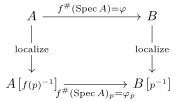Now we use locality in a key way to conclude $$\phi^{-1}(p) = f(p)$$ by commutativity: check that $$(f^\#_p)^{-1}({\mathfrak{m}}_p) = {\mathfrak{m}}_{f(p)} \xrightarrow{{\mathsf{loc}}^{-1}} f(p)$$, and $${\mathsf{loc}}^{-1}({\mathfrak{m}}_p) = p \xrightarrow{\phi} \phi(p)$$.

# 14 Monday, September 20

Last time: we proved the following:

1. If $$A\in \mathsf{Ring}$$, then $$(\operatorname{Spec}A, {\mathcal{O}}_{\operatorname{Spec}A})\in \mathsf{Loc}\mathsf{RingSp}$$.
2. If $$f\in \mathsf{CRing}(A, B)$$ is a ring morphism, it induces a morphism $$(f, f^\#) \in \mathsf{Loc}\mathsf{RingSp}(\operatorname{Spec}B, \operatorname{Spec}A)$$.
3. Moreover, every $$(f, f^\#) \in \mathsf{Loc}\mathsf{RingSp}(\operatorname{Spec}B, \operatorname{Spec}A)$$ is induced by some $$f\in {\mathsf{Top}}(A, B)$$.

Recall that a scheme $$(X, {\mathcal{O}}_X)$$ is a LRS which is locally isomorphic to some affine scheme $$(\operatorname{Spec}A, {\mathcal{O}}_{\operatorname{Spec}A})$$: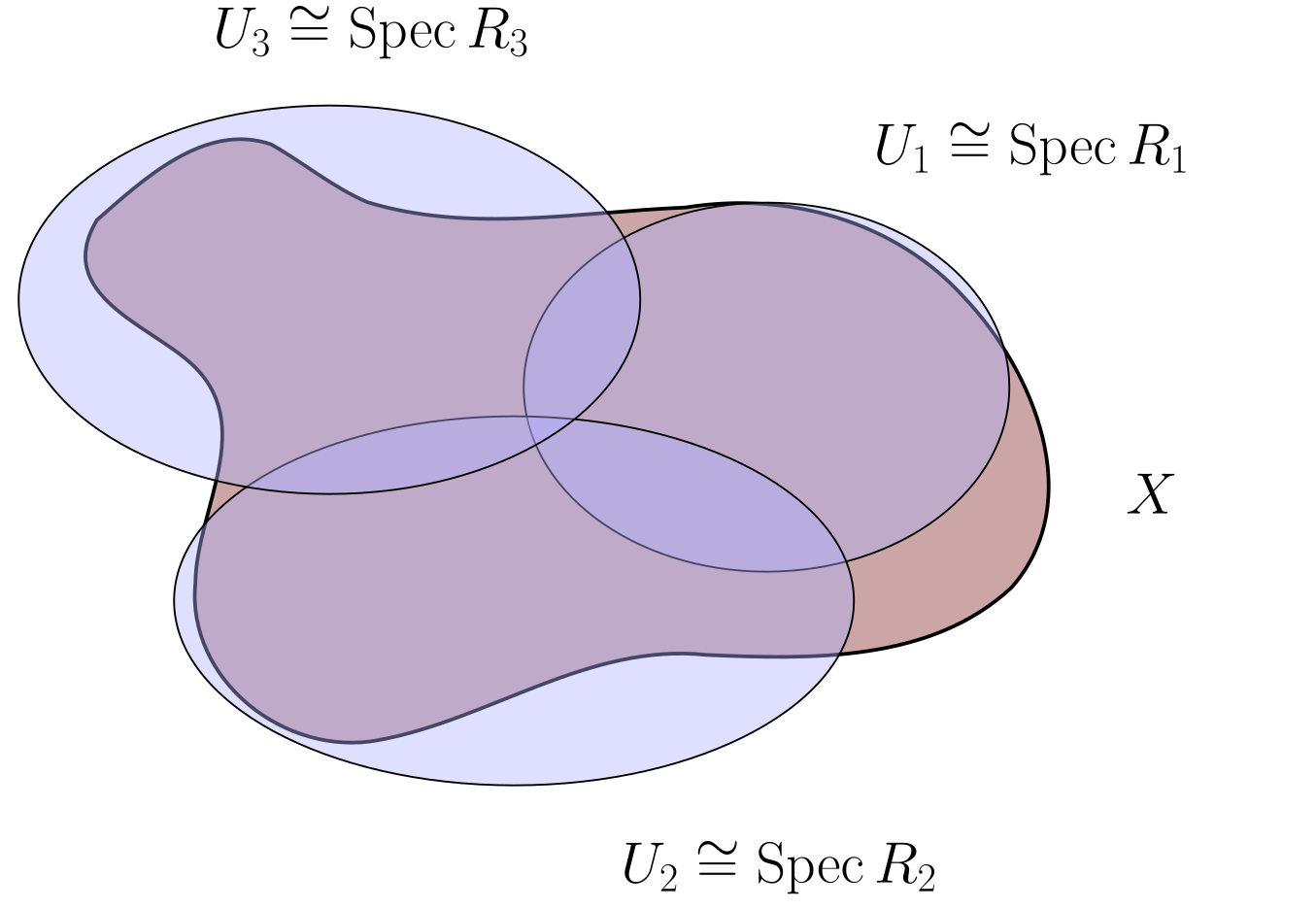A ring $$R$$ is complete with respect to $$I{~\trianglelefteq~}R$$ if $$R = \invlim_{\leftarrow} R/I^k$$. Elements can be written as sequence $$(a_k)_{k\geq 0}$$ such that $$a_k \equiv a_{k-1} \operatorname{mod}I^{k-1}$$.

A non-example: let $$R = {\mathbb{C}}\dagger{t}$$ and $$I = \left\langle{t}\right\rangle$$, then set

• $$a_0 = 1$$,
• $$a_1 = 1 + t$$,
• $$a_2 = 1 + t + t^2$$,
• $$a_3 = 1 + t + t^2 + t^3$$

This is an element in $$\colim_n R/I^n$$, but is not realized by any polynomial, since any polynomial is annihilated by quotienting by a large enough power of $$t$$. Note that $${\mathbb{C}} { \left[ {t} \right] } = {\mathbb{C}} { \left[ {[} \right] } t] { {}^{ \widehat{\left\langle{t}\right\rangle} } }$$.

Part (c) of the proposition would be false if we considered all ringed spaces. Let $$R \in \mathsf{DVR}$$, so $$R$$ is local with a principal maximal idea, or equivalently equipped with a valuation $$v: R\setminus\left\{{0}\right\}\to {\mathbb{Z}}_{\geq 0}$$ satisfying

• $$v(x+y) = v(x) + v(y)$$
• $$v(x+y) \geq \min{v(x), v(y)}$$

Then $$v^{-1}({\mathbb{Z}}_{\geq 0})$$ is the maximal ideal. Here we’ll additionally require that $$R$$ is complete with respect to its maximal ideal $${\mathfrak{m}}$$.

An example is $$R = {\mathbb{C}} { \left[ {t} \right] }$$, with $$v$$ the $$t{\hbox{-}}$$adic valuation. Another is $$R = { {\mathbb{Z}}_p }= {\mathbb{Z}}{ {}^{ \widehat{p} } }$$, the completion of $${\mathbb{Z}}$$ at the prime $$p$$, given by elements $$a_n\cdots a_0$$ with $$a_i \in \left\{{0, \cdots, p-1}\right\}$$. This has maximal ideal $${\mathfrak{m}}= p{ {\mathbb{Z}}_p }$$.

Let $$K = \operatorname{ff}(R)$$ be the fraction field of $$R$$, then \begin{align*} \operatorname{ff}({\mathbb{C}} { \left[ {t} \right] } ) = {\mathbb{C}} { \left( {t} \right) } = \left\{{\sum_{k\geq -N} a_k t^k {~\mathrel{\Big\vert}~}N\in {\mathbb{Z}}_{\geq 0} }\right\} .\end{align*} Also $$\operatorname{ff}({ {\mathbb{Z}}_p }) = { {\mathbb{Q}}_p }$$, and these are both examples of complete DVRs.

Consider $$(\operatorname{Spec}R, {\mathcal{O}}_{\operatorname{Spec}R})$$ and $$(\operatorname{Spec}K, {\mathcal{O}}_{\operatorname{Spec}K})$$. Then $$X_1\mathrel{\vcenter{:}}=\operatorname{Spec}R = \left\{{ \left\langle{0}\right\rangle, {\mathfrak{m}}}\right\}$$, and the closed sets are $$\emptyset, X_1, V({\mathfrak{m}}) = \left\{{{\mathfrak{m}}}\right\}$$. For $$X_2\mathrel{\vcenter{:}}=\operatorname{Spec}K = \left\{{\left\langle{0}\right\rangle}\right\}$$, there is one points since it’s a field. Use that $$\iota: R \hookrightarrow K$$, so define a morphism that does not come from a ring morphism $$R\to K$$: \begin{align*} (f, f^\#): (\operatorname{Spec}K, {\mathcal{O}}_{\operatorname{Spec}K}) &\to (\operatorname{Spec}R, {\mathcal{O}}_{\operatorname{Spec}R}) \\ \\ \\ f: \operatorname{Spec}K &\to \operatorname{Spec}R\\ 0 &\mapsto {\mathfrak{m}} \\ \\ f^\#: {\mathcal{O}}_{\operatorname{Spec}R} &\to f_* {\mathcal{O}}_{\operatorname{Spec}K} \\ \emptyset &\mapsto 0 \\ \operatorname{Spec}R & \mapsto R \\ \left\{{\left\langle{0}\right\rangle}\right\} &\mapsto K .\end{align*} using that $$f^{-1}(\left\langle{0}\right\rangle) = \left\langle{m}\right\rangle$$ and we can realize the last assignment as a distinguished open mapping to its stalk/localization. Then check \begin{align*} f_* {\mathcal{O}}_{\operatorname{Spec}K}(\emptyset) &= 0 f_* {\mathcal{O}}_{\operatorname{Spec}K}(\operatorname{Spec}R) &= K \\ f_* {\mathcal{O}}_{\operatorname{Spec}K}(\left\{{\left\langle{0}\right\rangle}\right\}) &= {\mathcal{O}}_{\operatorname{Spec}K}(f^{-1}(\left\langle{0}\right\rangle)) = {\mathcal{O}}_{\operatorname{Spec}K}(\emptyset) = 0 \\ .\end{align*} This would induces a commutative diagram, showing this is a morphism of ringed spaces:Is this a morphism of locally ringed spaces?

The answer is no, since the induced morphism on stalks won’t be morphisms of local rings. We can check \begin{align*} f^\#_{\left\langle{0}\right\rangle}: {\mathcal{O}}_{\operatorname{Spec}R, {\mathfrak{m}}} &\to {\mathcal{O}}_{\operatorname{Spec}K, \left\langle{0}\right\rangle} \\ f^\#_{\left\langle{0}\right\rangle}: R = R \left[ { \scriptstyle { {{\mathfrak{m}}}^{-1}} } \right] &\to K \left[ { \scriptstyle { {\left\langle{0}\right\rangle}^{-1}} } \right] = K ,\end{align*} and $$(f^\#_{\left\langle{0}\right\rangle})^{-1}(\left\langle{0}\right\rangle) = \left\langle{0}\right\rangle \neq {\mathfrak{m}}$$, which is not the maximal ideal of $$R$$.

On the other hand, using part (b) of the proposition, any $$\phi\in \mathsf{Ring}(R, K)$$ induces a morphism $$\tilde \phi: \mathsf{Loc}\mathsf{RingSp}(\operatorname{Spec}K, \operatorname{Spec}R)$$. So $$(f, f^\#)$$ is not induced by any such ring map $$\phi$$.

So the functor \begin{align*} \mathsf{Ring}&\to \mathsf{RingSp}\\ A &\mapsto (\operatorname{Spec}A, {\mathcal{O}}_{\operatorname{Spec}A}) \end{align*} is not fully faithful, but restricting the essential image to $$\mathsf{Loc}\mathsf{RingSp}$$.

Consider the heuristic $$\operatorname{Spec}{\mathbb{C}} { \left[ {t} \right] } \sim {\mathbb{D}}\subseteq {\mathbb{C}}$$ and $$\operatorname{Spec}{\mathbb{C}} { \left( {t} \right) } \sim {\mathbb{D}}\setminus\left\{{0}\right\}$$.

# 15 Wednesday, September 22

Today: how to build more schemes by gluing known ones together. Let $$(X_1, {\mathcal{O}}_{X_1})$$ and $$(X_2, {\mathcal{O}}_{X_2}) \in {\mathsf{Sch}}$$, i.e. locally ringed spaces locally isomorphic to $$(\operatorname{Spec}R, {\mathcal{O}}_{\operatorname{Spec}R})$$. Let $$U_i \subseteq X_i$$, and suppose we’re given an isomorphism in $$\mathsf{Loc}\mathsf{RingSp}$$: \begin{align*} \phi: (U_1, { \left.{{{\mathcal{O}}_{X_1}}} \right|_{{U_1}} }) \to (U_2, { \left.{{{\mathcal{O}}_{X_2}}} \right|_{{U_2}} }) .\end{align*}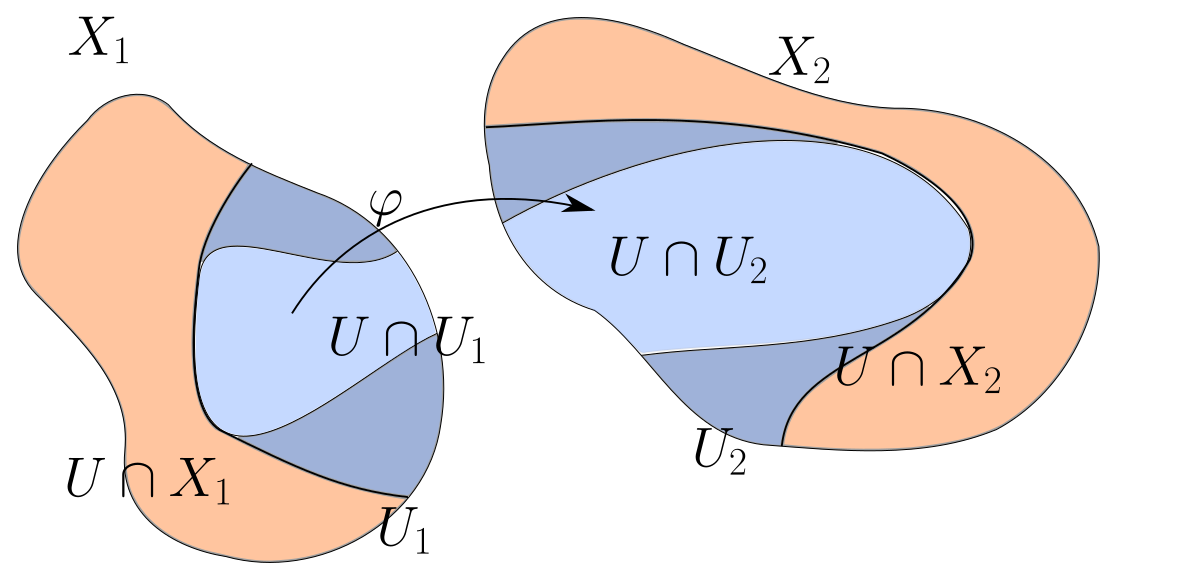Define a locally ringed space as follows: set $$X = X_1 {\textstyle\coprod}X_2/ x\sim \phi(x)$$, and define \begin{align*} {\mathcal{O}}_X(U) \mathrel{\vcenter{:}}= \left\{{ s_1 \in {\mathcal{O}}_{X_1}(X_1 \cap U), s_2\in {\mathcal{O}}_{X_2}(X_2 \cap U) {~\mathrel{\Big\vert}~}{ \left.{{s_1}} \right|_{{U_1 \cap U}} } = \phi^\#(U_2 \cap U)\qty{ { \left.{{s_2}} \right|_{{U_2 \cap U}} } } }\right\} .\end{align*}

Consider $$I \mathrel{\vcenter{:}}=(0, 1) \subseteq {\mathbb{R}}$$ and take $$X_1 = X_2 \mathrel{\vcenter{:}}=(I, C^\infty({-}; {\mathbb{R}}))$$. Using these to cover the circle, we can obtain $$(S^1, C^\infty({-}; {\mathbb{R}}))$$, using smooth functions that agree on the overlap (here a disjoint union of smaller intervals).

Let $$X_1 = X_2 \mathrel{\vcenter{:}}={\mathbb{A}}^1_{/ {k}} \mathrel{\vcenter{:}}=\operatorname{Spec}k[x]$$, and set $$U_1 \subseteq X_1 \mathrel{\vcenter{:}}= D(x)$$. Then take the clear isomorphism \begin{align*} (U_1, {\mathcal{O}}_{X_1}{ \left.{{}} \right|_{{U_1}} }) \xrightarrow{\operatorname{id}} (U_2, {\mathcal{O}}_{X_2}{ \left.{{}} \right|_{{U_2}} }) ,\end{align*} since they’re the same open subset of the same affine variety. Gluing yields the following: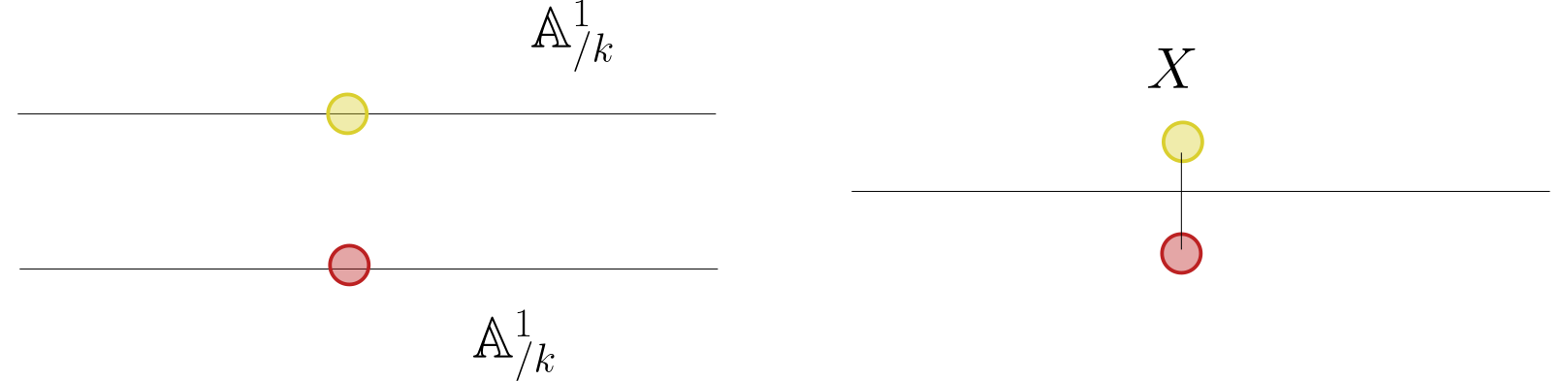Prove this is not an affine scheme. Use that regular functions are determined by their values on a Zariski open.

For $$X = \operatorname{Spec}R$$, recall that $$D(f) \mathrel{\vcenter{:}}=\left\{{ p\in \operatorname{Spec}R {~\mathrel{\Big\vert}~}p\not\ni f}\right\}$$. Then $$(D(f), { \left.{{ {\mathcal{O}}_X }} \right|_{{D(f)}} })$$ is an affine scheme.

Consider $$R \left[ { { {f}^{-1}} } \right] \mathrel{\vcenter{:}}=\left\{{r/f^k {~\mathrel{\Big\vert}~}r\in R, k\geq 0}\right\}/\sim$$. There’s a map $$R\to R \left[ { { {f}^{-1}} } \right]$$ which induces a map $$\operatorname{Spec}R \left[ { { {f}^{-1}} } \right] \to \operatorname{Spec}R$$, and we claim it’s the inclusion of $$D(f)$$.

$$\operatorname{Spec}R \left[ { { {f}^{-1}} } \right] = D(f)$$ as spaces.

This uses that primes in the localization are primes in $$R$$ not intersecting the inverted set. So $$\operatorname{Spec}R \left[ { { {f}^{-1}} } \right] = \left\{{p\in \operatorname{Spec}R {~\mathrel{\Big\vert}~}p \cap\left\langle{f}\right\rangle = \emptyset}\right\}$$, then use that $$p \cap\left\{{f^k}\right\} = \emptyset \iff f\not\in p$$, since prime ideals are radical. We now want to show $${\mathcal{O}}_{\operatorname{Spec}R \left[ { { {f}^{-1}} } \right]} = { \left.{{{\mathcal{O}}_{\operatorname{Spec}R}}} \right|_{{D(f)}} }$$. Check that \begin{align*} {\mathcal{O}}_{\operatorname{Spec}R \left[ { \scriptstyle { {f}^{-1}} } \right]} = \left\{{s:U\to \displaystyle\coprod_{p\in U} (R \left[ { \scriptstyle { {f}^{-1}} } \right]) \left[ { \scriptstyle { {p}^{-1}} } \right]}\right\} \end{align*} and \begin{align*} { \left.{{{\mathcal{O}}_{\operatorname{Spec}R}}} \right|_{{D(f)}} }(U) = \left\{{s: U \to \displaystyle\coprod_{p\in U} R \left[ { \scriptstyle { {p}^{-1}} } \right]}\right\} ,\end{align*} but $$(R_f)_p = R_p$$ since $$f\not \in p$$. This uses that $$(R \left[ { { {S_1}^{-1}} } \right]) \left[ { { {S_2}^{-1}} } \right] = R \left[ { { {S_2}^{-1}} } \right]$$ when $$S_1 \subseteq S_2$$. The first are functions of the form $$(a/f^k)/(b/f^\ell) = f^\ell a/f^k b$$, so anything locally a fraction in $$R_f$$ is locally a fraction in $$R$$.

Let $$X_1 = {\mathbb{A}}^1_{/ {k}}$$ with $$U_1 \mathrel{\vcenter{:}}= D(x) \subseteq X_2$$ and $$X_2 = {\mathbb{A}}^1_{/ {k}}$$ with $$U_2 = D(y)$$. Then \begin{align*} (U_1, {\mathcal{O}}_{X_1}{ \left.{{}} \right|_{{U_1}} }) \cong (k[x, x^{-1}], {\mathcal{O}}_{\operatorname{Spec}k[x, x^{-1}]}) \xrightarrow{(\phi, \phi^\#)} (U_2, {\mathcal{O}}_{X_2}{ \left.{{}} \right|_{{U_2}} }) \cong (k[y, y^{-1}], {\mathcal{O}}_{\operatorname{Spec}k[y, y^{-1}]}) .\end{align*}

Then $$(\phi, \phi^\#)$$ is an isomorphism in $$\mathsf{Loc}\mathsf{RingSp}$$ is given by a unique isomorphism \begin{align*} \iota: k[x, x^{-1}] &\to k[y, y^{-1}] \\ y &\mapsto x \\ y^{-1}&\mapsto x^{-1} .\end{align*} Note that there is another isomorphism: \begin{align*} \iota': k[x, x^{-1}] &\to k[y, y^{-1}] \\ y &\mapsto x^{-1}\\ y^{-1}&\mapsto x .\end{align*} So glue instead by the morphism $$(\phi, \phi^\#)$$ induced by $$\iota'$$. We’ll then define projective space as \begin{align*} {\mathbb{P}}^1_{/ {k}} \mathrel{\vcenter{:}}={\mathbb{A}}^1_{/ {k}} \displaystyle\coprod_{(\phi, \phi^\#)} {\mathbb{A}}^1_{/ {k}} .\end{align*} Note that this works over any ring!

What does this do to closed points? The closed points of $$\operatorname{Spec}k[x, x^{-1}]$$ are $$\left\{{(x-c) {~\mathrel{\Big\vert}~}c\neq 0}\right\}$$ if $$k=\mkern 1.5mu\overline{\mkern-1.5muk\mkern-1.5mu}\mkern 1.5mu$$, which corresponded to the closed points $$c\in {\mathbb{A}}^1_{/ {k}}$$ as a variety. Under $$x\mapsto y^{-1}$$, we have $$(x-c)\mapsto (y^{-1}- c) = (y-c^{-1})$$, thus the variety point $$c$$ gets sent to $$c^{-1}$$.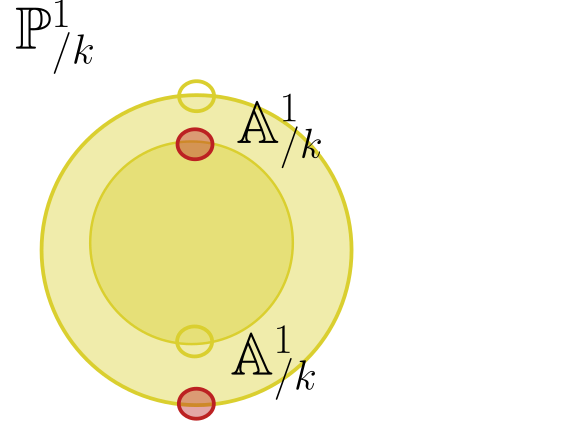# 16 Projective Varieties (Tuesday, September 28)

## 16.1 Projective Space

Let $$R$$ be a ring, then the affine space of dimension $$n$$ over $$R$$ is defined as \begin{align*} {\mathbb{A}}^n_{/ {R}} \mathrel{\vcenter{:}}=(\operatorname{Spec}S, {\mathcal{O}}_{\operatorname{Spec}S}) && S \mathrel{\vcenter{:}}= R[{ {x}_1, {x}_2, \cdots, {x}_{n}}] .\end{align*}

Let $$S\in {\mathsf{Sch}}$$, then $$X\in {\mathsf{Sch}}_{/ {S}}$$ is a scheme over $$S$$ iff $$X$$ is a scheme equipped with a morphism of schemes $$X\to S$$. These form a category where morphisms $$\phi$$ are commuting triangles: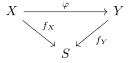Since $${\mathbb{Z}}\in \mathsf{CRing}$$ is initial, there exists a unique ring morphism $${\mathbb{Z}}\to {\mathbb{R}}$$ for any $$R\in \mathsf{CRing}$$. Similarly, $$\operatorname{Spec}{\mathbb{Z}}\in{\mathsf{Sch}}$$ is final, so there exist unique morphisms $$\operatorname{Spec}R\to \operatorname{Spec}{\mathbb{Z}}$$ for every $$R$$, and thus $${\mathsf{Sch}}_{/ {\operatorname{Spec}{\mathbb{Z}}}} \cong {\mathsf{Sch}}$$ just recovers the category of schemes.

Recall that if $$k = \mkern 1.5mu\overline{\mkern-1.5muk\mkern-1.5mu}\mkern 1.5mu \in \mathsf{Field}$$ is algebraically closed, then $${\mathbb{P}}^n_{/ {k}} \mathrel{\vcenter{:}}={\mathbb{A}}^{n+1}_{/ {k}} \setminus\left\{{0}\right\}/\sim$$ where $$\mathbf{x}\sim \lambda \mathbf{x}$$ for every $$\lambda \in k^{\times}$$, or equivalently $${\operatorname{Gr}}_k(k^{n+1})$$, the space of lines through the origin in $$k^{n+1}$$ (regarded as a vector space). Let $$f\in k[x_1, \cdots, x_{n}]_d^{ { \mathrm{homog} } }$$ be homogeneous of degree $$d$$, so $$f(\lambda \mathbf{x}) = \lambda^d f(\mathbf{x})$$. Then its vanishing locus in projective space is well-defined: \begin{align*} V_p(f) \mathrel{\vcenter{:}}=\left\{{ \mathbf{x} = {\left[ {x_0: \cdots : x_n} \right]} \subseteq {\mathbb{P}}^n_{/ {k}} {~\mathrel{\Big\vert}~}f(\mathbf{x}) = 0 }\right\}\subseteq {\mathbb{P}}^n_{/ {k}} .\end{align*}

## 16.2 Graded Rings and Homogeneous Ideals

A ring $$R\in \mathsf{CRing}$$ is graded if it admits a decomposition as an abelian group $$R + \bigoplus _{d\geq 0} S_d$$, where $$S_d$$ are the degree $$d$$ pieces satisfying $$S_a + S_b \subseteq S_{a+b}$$.

Note that $$S_d \in {\mathsf{S_0}{\hbox{-}}\mathsf{Mod}}$$ for any $$S \in {\mathsf{gr}\,}\mathsf{CRing}$$ and any degree $$d$$.

$$R\mathrel{\vcenter{:}}= k[x_1, \cdots, x_{n}]^ { \mathrm{homog} }$$ is graded by monomial degree: \begin{align*} 1 + (x_0) + (x_0^2 +x_1^2) \in R_0 + R_1 + R_2 .\end{align*}

Let $$S\in {\mathsf{gr}\,}\mathsf{CRing}$$ be a graded ring, then an ideal $$I {~\trianglelefteq~}S$$ is homogeneous if

1. $$I$$ is generated by homogeneous elements, and
2. For all $$f\in I$$, all homogeneous pieces $$f_i \in S_i$$ such that $$f = \sum_{i\leq d} f_d$$ lie in $$I$$.

If $$f \mathrel{\vcenter{:}}= 1 + x_0 + x_0^2 + x_1^2 \in I$$ is an element in a homogeneous ideal, then $$1, x_0, x_0^2 + x_1\in I$$ as well.

If $$I{~\trianglelefteq~}k[x_1, \cdots, x_{n}]$$ is a homogeneous ideal, say $$I = \left\langle{{ {f}_1, {f}_2, \cdots, {f}_{m}}}\right\rangle$$ with each $$f_i$$ homogeneous of uniform degree $$d$$, then $$V_p(I)$$ is a well-defined projective variety.

• Take $$V_p(y^2 - (x^3 + axz^2 + bz^3))$$ for $$a, b\in k$$ and $$4a^3-27b^2 \neq 0$$. This defines an elliptic curve.

• $${\mathbb{P}}^n_{/ {k}} = V_p(0)$$.

We define $$S_+ \mathrel{\vcenter{:}}=\bigoplus _{d\geq 1} S_d$$ to be the irrelevant ideal.

## 16.3 Projective Nullstellensatz

We again define the Zariski topology on $$X = V_p(I)$$ whose closed sets are of the form $$V_p(J)$$ for $$J \subseteq (k[x_1, \cdots, x_{n}]/I)^{ { \mathrm{homog} } }$$

Let $$k[X] \mathrel{\vcenter{:}}= k[x_1, \cdots, x_{n}]/I$$ be the projective coordinate ring of $$X \subset {\mathbb{P}}^n_{/ {k}}$$ and $$I = I(X)$$. Then there is a bijection: \begin{align*} \left\{{\substack{ \text{Points }x\in X }}\right\} &\rightleftharpoons \left\{{\substack{ \text{Homogeneous }I\in \operatorname{mSpec}S \\ \text{with }I\not\supseteq S_+ }}\right\} \\ x &\mapsto I(x) \mathrel{\vcenter{:}}=\left\langle{ f\in k[x_1, \cdots, x_{n}]^ { \mathrm{homog} } {~\mathrel{\Big\vert}~}f(x) = 0}\right\rangle \\ V_p(I) &\mapsfrom I \end{align*}

Note $$I$$ doesn’t contain $$S_+$$ iff $$I$$ is strictly contained in $$S_+$$.

## 16.4 Proj

Let $$S\in {\mathsf{gr}\,}\mathsf{CRing}$$, then define \begin{align*} \mathop{\mathrm{Proj}}S \mathrel{\vcenter{:}}=\left\{{p\in \operatorname{Spec}S \text{ homogeneous} {~\mathrel{\Big\vert}~}p\not\supseteq S_+}\right\} ,\end{align*} where $$S_+ \mathrel{\vcenter{:}}=\bigoplus_{d\geq 1} S_d$$ is the irrelevant ideal.

We’ll define $${\mathcal{O}}_{\mathop{\mathrm{Proj}}S}$$ next class.

# 17 Friday, October 01

Recall the Proj construction: for $$S = \bigoplus_{d\geq 0} S_d \in {\mathsf{gr}\,}\mathsf{CRing}$$ we define the irrelevant ideal $$S_+ \mathrel{\vcenter{:}}=\bigoplus _{d\geq 1} S_d$$ and \begin{align*} \mathop{\mathrm{Proj}}S &\mathrel{\vcenter{:}}=\left\{{p\in \operatorname{Spec}S \text{ homog } {~\mathrel{\Big\vert}~}p\not\supseteq S_+}\right\} \\ {\mathcal{O}}_{\mathop{\mathrm{Proj}}S} &\mathrel{\vcenter{:}}=\left\{{s: U\to \displaystyle\coprod_{p\in U} S \left[ { \scriptstyle { { \qty{ {p}^c } }^{-1}} } \right] {~\mathrel{\Big\vert}~}s(p) \in S \left[ { \scriptstyle { { \qty{ {p}^c } }^{-1}} } \right], s \text{ locally a fraction}}\right\} ,\end{align*} recalling that $$S \left[ { { { \qty{ {p}^c } }^{-1}} } \right] = \left\{{a /f {~\mathrel{\Big\vert}~}\deg a = \deg f, a,f\in S, f\not\in p}\right\}$$. We showed this was a locally ringed space using \begin{align*} ( D(f), { \left.{{{\mathcal{O}}_{\mathop{\mathrm{Proj}}S}}} \right|_{{D(f)}} } { { \, \xrightarrow{\sim}\, }}(\operatorname{Spec}S \left[ { \scriptstyle { {f}^{-1}} } \right], {\mathcal{O}}_{\operatorname{Spec}S \left[ { \scriptstyle { {f}^{-1}} } \right]}) ,\end{align*} where $$D(f) \mathrel{\vcenter{:}}=\left\{{p\in \mathop{\mathrm{proj}}S {~\mathrel{\Big\vert}~}f\not\in p}\right\}$$, and thus $$\mathop{\mathrm{Proj}}S \in {\mathsf{Sch}}$$.

Check that there is a natural map of schemes $$\mathop{\mathrm{Proj}}S\to \operatorname{Spec}S_0$$.

Consider \begin{align*} {\mathbb{P}}^n_{/ {R}} \mathrel{\vcenter{:}}=\mathop{\mathrm{Proj}}R[x_0,\cdots, x_n] && R = k = \mkern 1.5mu\overline{\mkern-1.5muk\mkern-1.5mu}\mkern 1.5mu \in \mathsf{Field} .\end{align*} Then the closed points of $${\mathbb{P}}^n_{/ {k}}$$ are of the form $$\left\langle{a_i x_j - a_j x_i}\right\rangle \in \operatorname{mSpec}k[x_1, \cdots, x_{n}]$$ for points $$[a_0: \cdots : a_n] \in k^n/\sim$$ where $$\mathbf{a} \sim \lambda \mathbf{a}$$ for $$\lambda \in k^{\times}$$. Note that $$D(x_i) = \left\{{p\in {\mathbb{P}}^n_{/ {k}} {~\mathrel{\Big\vert}~}x_i \not\in p}\right\}$$ – what are the closed points? We discard the hyperplane $$a_i = 0$$ in $${\mathbb{P}}^n$$ to obtain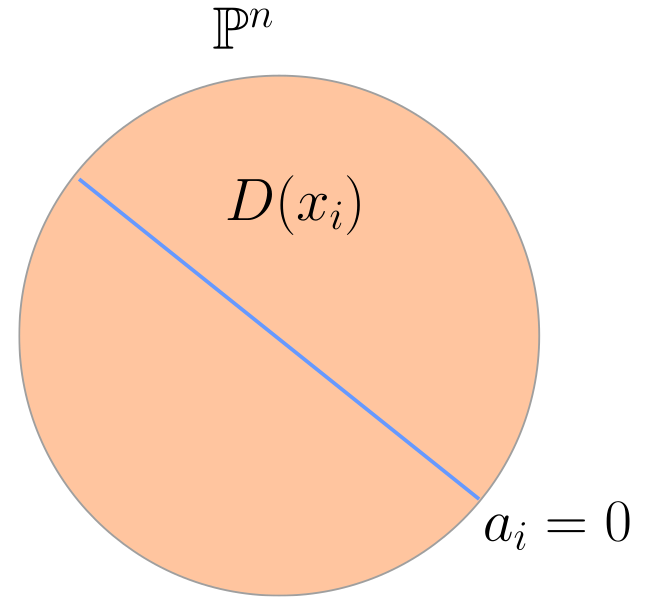Then $$x_i \in {\mathfrak{m}}_q$$ for $$q \mathrel{\vcenter{:}}=[a_0: \cdots : a_n]$$ iff $$a_i=0$$, and \begin{align*} D(x_i) &= \operatorname{Spec}k[x_1, \cdots, x_{n}] \left[ { \scriptstyle { {x_i}^{-1}} } \right] \\ &= \left\{{f(x_0, \cdots, x_n)/x_i^d {~\mathrel{\Big\vert}~}\deg f = d}\right\} \\ &= \left\{{f\qty{ {x_0\over x_i }, \cdots, 1, \cdots, {x_n \over x_i }}}\right\} \\ &= k { \left[ {{x_0\over x_i}, \cdots, {x_n \over x_i} } \right] } \\ &\cong {\mathbb{A}}^n_{/ {k}} .\end{align*} We claim that $$\displaystyle\bigcup_{i\geq 0} D(x_i) = {\mathbb{P}}^n_{/ {k}}$$, or equivalently $$\emptyset = \displaystyle\bigcap_{i \geq 0} V(x_i) = V(\left\langle{x_0,\cdots, x_n}\right\rangle)$$. But this is true since $$\left\langle{x_0, \cdots, x_n}\right\rangle = S^+$$ is the irrelevant ideal.

Let $$k = \mkern 1.5mu\overline{\mkern-1.5muk\mkern-1.5mu}\mkern 1.5mu \in \mathsf{Field}$$. Then there is a fully faithful embedding of categories \begin{align*} F: {\mathsf{Var}}_{/ {k}} \hookrightarrow{\mathsf{Sch}}_{/ {k}} .\end{align*} Here $${\mathsf{Var}}_{/ {k}}$$ are defined as topological spaces with sheaves of rings of regular functions which admitted an affine cover of the form $$V(I) \subseteq {\mathbb{A}}^n_{/ {k}}$$ (viewed as a variety).

Consider $$X\mathrel{\vcenter{:}}={\mathbb{A}}^2_{/ {k}}$$ as a variety and separately as a scheme $$X'$$. As a variety, $$X \mathrel{\vcenter{:}}= k{ {}^{ \scriptscriptstyle\times^{2} } }$$ with the Zariski topology, while as a scheme $$X' = \operatorname{Spec}k[x, y]$$ with the Zariski topology. Then there is an inclusion $$X \hookrightarrow X'$$ which is a bijection on closed points.

More generally, for $$X\in {\mathsf{Top}}$$ any space, define $$t(X)$$ to be the set of irreducible closed subsets. Some facts:

• For $$Y \subseteq X$$ closed, $$t(Y) \subseteq t(X)$$,
• $$t(Y_1 \cup Y_2) = t(Y_1) \cup t(Y_2)$$,
• $$t( \displaystyle\bigcap_i Y_i) = \displaystyle\bigcap_i t(Y_i)$$.

Define a topology on $$t(X)$$ by declaring closed sets to be any of the form $$t(Y)$$ for $$Y \subseteq X$$ closed. Note that the scheme $$X'$$ has non-closed points, i.e. irreducible subvarieties, i.e. irreducible closed subsets of $$X$$ as a variety: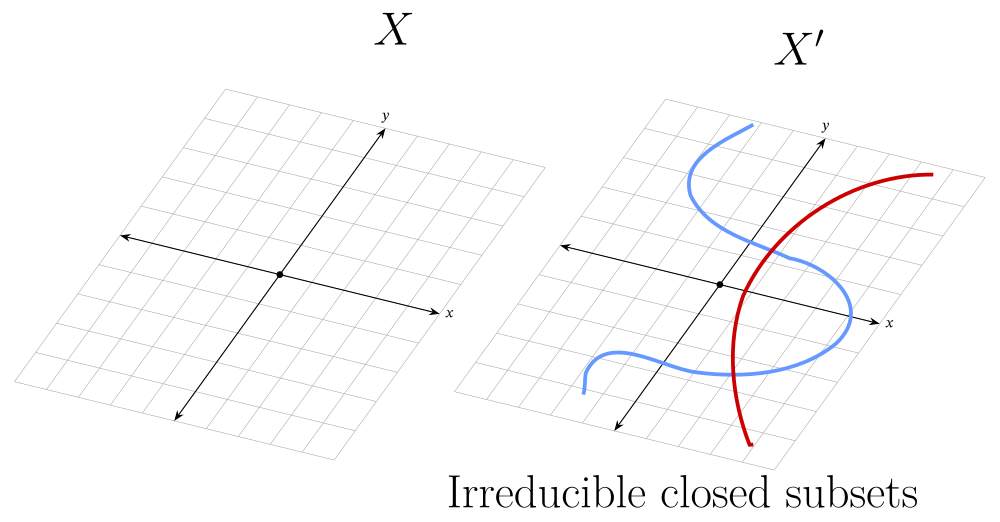Then consider the map \begin{align*} \alpha: X &\to t(X) \\ p &\mapsto \left\{{p}\right\} ,\end{align*} noting that this is only well-defined if points are closed in $$X$$.

Now let $$V\in {\mathsf{Var}}_{/ {k}}$$ with its sheaf of regular functions $${\mathcal{O}}_V$$ (i.e. restrictions of polynomials). Define a sheaf of rings on $$t(V)$$ as $$\alpha_* {\mathcal{O}}_V$$, using that $$\alpha$$ is continuous, and noting that $$\alpha^{-1}(U) = U \cap\alpha(X)$$.

To see this is a scheme, it suffices to check for $$V$$ affine since this entire construction is compatible with restriction and we can take an affine cover. Letting $$I = I(V)$$ for $$V\in {\mathsf{Aff}}{\mathsf{Var}}_{/ {k}}$$, then $$(t(V), \alpha_* {\mathcal{O}}_V){ { \, \xrightarrow{\sim}\, }}\operatorname{Spec}k[V] \mathrel{\vcenter{:}}=\operatorname{Spec}k[x_1, \cdots, x_{n}]/I$$. There is a bijection \begin{align*} t(V) &\rightleftharpoons\operatorname{Spec}k[V] \\ Y &\mapsto I(Y) \\ V(p) &\mapsfrom p .\end{align*} One can check that the topology on $$t(V)$$ bijects with the Zariski topology on $$\operatorname{Spec}k[V]$$, and \begin{align*} \alpha_* {\mathcal{O}}_V(t(V)) = {\mathcal{O}}_V(V) = {\mathcal{O}}_{\operatorname{Spec}k[V]}(\operatorname{Spec}k[V]) = k[V] .\end{align*}

Check this on open subsets of $$t(V)$$.

$${\mathcal{O}}_X \in {\mathsf{Sh}}(X, {\mathsf{Alg}_{/k} })$$ being a sheaf of $$k{\hbox{-}}$$algebras means the following diagram commutes:This is the data of a morphism $$(X, {\mathcal{O}}_X) \to \operatorname{Spec}k$$.

What’s the point of the theorem? Not everything of geometric interest is in the essential image of $$F$$. Consider $$V(y-x^2) \subseteq {\mathbb{A}}^2_{/ {k}}$$, and consider intersecting it with lines $$y=t$$: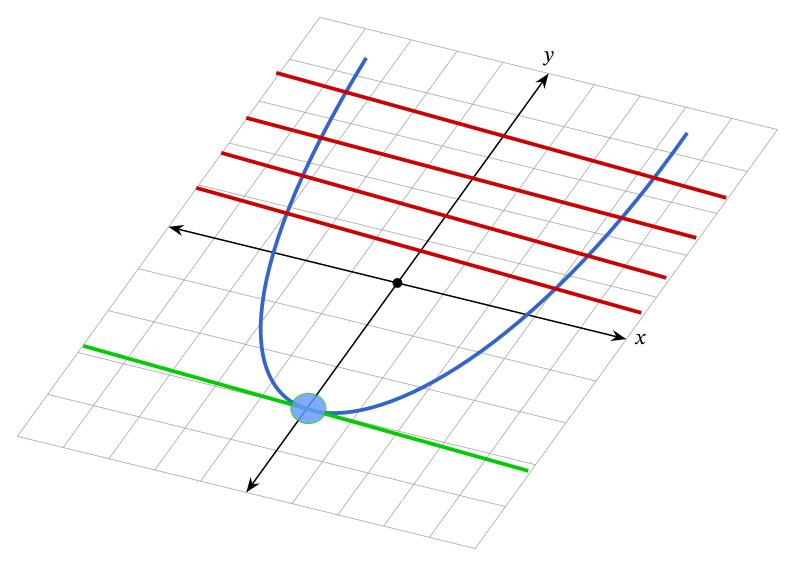Letting $$I_1 = I_1(\left\langle{y-x^2}\right\rangle)$$ and $$I_2 = I(\left\langle{y-t}\right\rangle)$$, intersecting in varieties yields \begin{align*} V_1 \cap V_2 = V(I_1 + I_2) = V(\sqrt{I_1 + I_2}) .\end{align*} One can check $$I_1 + I_2 = (x-\sqrt t, y-t)\cdot (x+\sqrt t, y-t)$$, and $$\operatorname{Spec}k[x, y] / \left\langle{y-x^2, y}\right\rangle = \operatorname{Spec}k[x]/\left\langle{x^2}\right\rangle$$ when $$t=0$$ (i.e. when there’s a tangency with multiplicity), since the scheme intersection is $$\operatorname{Spec}k[x, y] / \left\langle{I_1 + I_2}\right\rangle$$. Note that the regular functions on a point are just constant, so the sheaf of regular functions on a point is $$k$$ itself and thus doesn’t pick up the multiplicity of the intersection.

There can be issues for $$\operatorname{Spec}R$$ when $$R$$ is finitely generated but not reduced!

# 18 Monday, October 04

We’ll set up some properties for schemes. A scheme can be:

• Irreducible
• Smooth
• Reduced
• Noetherian

Recall that $$X\in {\mathsf{Top}}$$ is connected iff whenever $$X = X_1 {\textstyle\coprod}X_2$$ with $$X_i$$ closed, one of $$X_i = X$$ and the other is empty. $$X$$ is irreducible iff this holds in the weaker case when $$X = X_1 \cup X_2$$ is not necessarily disjoint. Note that irreducible implies connected.

$$(X, {\mathcal{O}}_X) \in {\mathsf{Sch}}$$ is connected (resp. irreducible) iff $${ {\left\lvert {X} \right\rvert} } \in {\mathsf{Top}}$$ is connected (resp. irreducible).

$$X\mathrel{\vcenter{:}}={\mathbb{A}}^n_{/ {k}}$$ is connected and irreducible. Write $${\mathbb{A}}^n = \operatorname{Spec}k[x_1, \cdots, x_{n}]$$, whose closed sets are $$V(I) \mathrel{\vcenter{:}}=\left\{{p\supseteq I}\right\}$$. Suppose $$X = V(I) \cup V(J) = V(IJ)$$, then all primes $$p$$ satisfy $$IJ \supseteq p$$, and this $$IJ \supseteq\cap_{p\in \operatorname{Spec}R} p = {\sqrt{0_{R}} } = 0$$, using a fact from commutative algebra. Then $$IJ = 0$$ implies $$I=0$$ (wlog), so $$V(I) = X$$.

Let $$X \mathrel{\vcenter{:}}=\operatorname{Spec}k[x, y] / \left\langle{xy}\right\rangle$$, for $$k$$ not necessarily algebraically closed. This is roughly the coordinate axis in $$k^2$$, except there are generic points corresponding to irreducible closed subsets. Points $$\left\langle{x-a, y-b}\right\rangle$$ are closed and contained in $$X$$ when $$(a, b)$$ is on the axis. There are non-maximal primes $$\left\langle{x}\right\rangle, \left\langle{y}\right\rangle$$.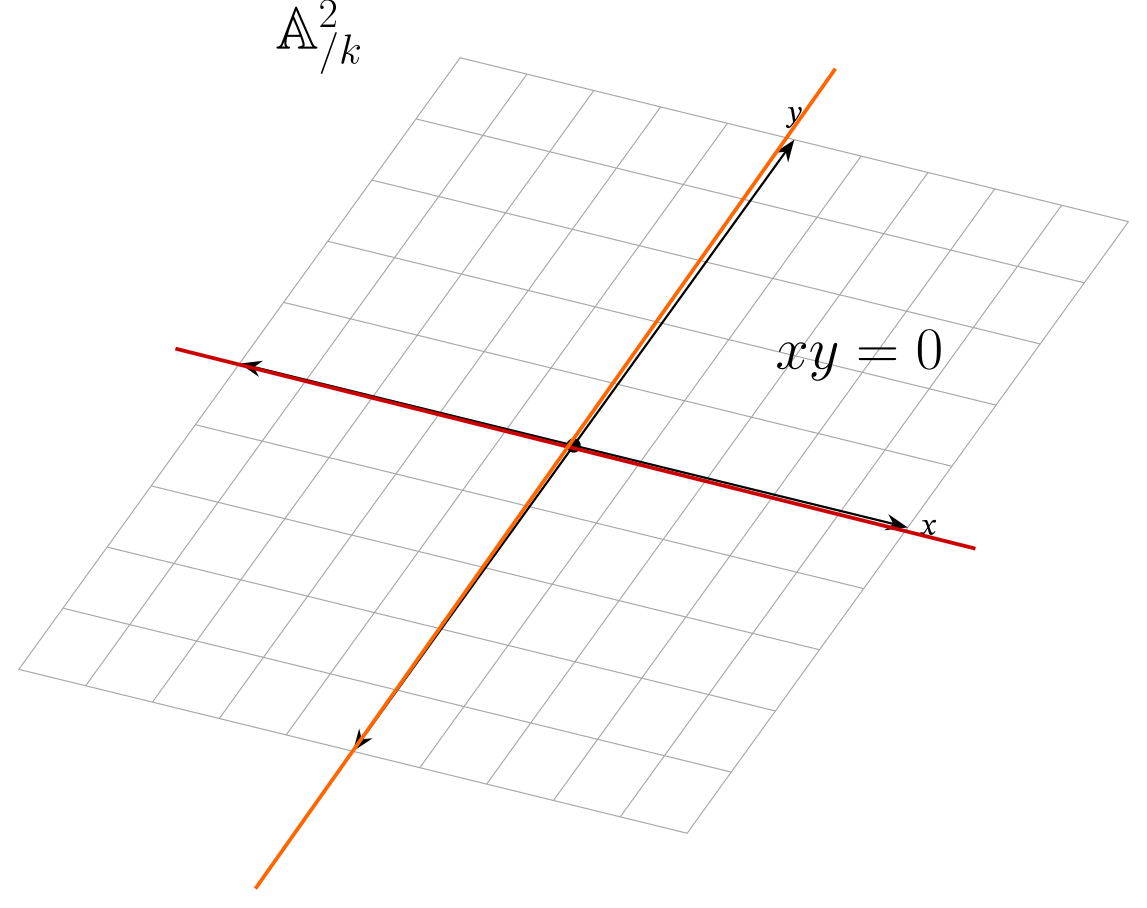Note $$X \supseteq V(\left\langle{x}\right\rangle) = \left\{{p\in x}\right\} = \left\{{\left\langle{x}\right\rangle}\right\} \cup\left\{{\left\langle{x, y-b}\right\rangle}\right\}$$, and similarly, $$V(\left\langle{y}\right\rangle) = \left\{{\left\langle{y}\right\rangle}\right\} \cup\left\{{\left\langle{x-a, y}\right\rangle}\right\}$$. However $$V(x) \cup V(y) = X$$ but $$V(x) \cap V(y) = \left\{{\left\langle{x, y}\right\rangle}\right\}$$, making $$X$$ connected but not irreducible.

Let $$X\mathrel{\vcenter{:}}=\operatorname{Spec}( k[x]/\left\langle{x(x-1)}\right\rangle ) \cong \operatorname{Spec}( k[x]/\left\langle{x}\right\rangle ) \oplus \operatorname{Spec}(k[x]/\left\langle{x-1}\right\rangle) \cong \operatorname{Spec}(k \oplus k)$$, using the Chinese remainder theorem. Note that this has two prime ideals, $$\left\{{0 \oplus k, k \oplus 0}\right\}$$, making it a discrete space and thus a disjoint union of its two closed points. Note that $$0 \oplus 0$$ isn’t prime, consider $$(a, 0) \oplus (0, b)$$.

Consider $$X \mathrel{\vcenter{:}}=\operatorname{Spec}{ {\mathbb{Z}}_p }= \left\{{ \left\langle{0}\right\rangle, \left\langle{p}\right\rangle}\right\}$$. Then $${ \operatorname{cl}} _X\qty{ \left\{{\left\langle{0}\right\rangle}\right\}} = X$$, since $$0$$ is not a closed point. This is connected and irreducible, and the picture is a point with a fuzzy point:Show $$\operatorname{Spec}{\mathbb{Z}}$$ is connected and irreducible.

$$(X, {\mathcal{O}}_X) \in {\mathsf{Sch}}$$ is reduced iff $${\mathcal{O}}_X(U)$$ is a reduced ring for all open $$U \subseteq X$$, i.e. contains no nilpotents. Equivalently, $${\mathcal{O}}_{X, p}$$ is reduced for all $$p\in X$$.

Note that this is a genuinely new concepts for schemes, since functions valued in a field always yields a reduced ring.

Let $$R$$ be reduced and take $$X\mathrel{\vcenter{:}}=\operatorname{Spec}R$$, then for $$U \subseteq X$$ with $$U = V(I)^c \supseteq D(f) \mathrel{\vcenter{:}}= V(f)$$ for any $$f\in I$$. In fact $$U = \displaystyle\bigcup_{f\in I} D(f)$$, and $${\mathcal{O}}_X(U)$$ by the sheaf property can be written as \begin{align*} {\mathcal{O}}_X(U) &= \left\{{\phi_f \in {\mathcal{O}}_X(D(f)) {~\mathrel{\Big\vert}~}{ \left.{{\phi_f}} \right|_{{D(f) \cap D(g) }} } = { \left.{{\phi_f}} \right|_{{D(f) \cap D(g)}} } }\right\} \\ &\subseteq \prod_{f\in I} {\mathcal{O}}_X(D(f)) \\ &= \prod_{f\in I} R \left[ { \scriptstyle { {f}^{-1}} } \right] ,\end{align*} by the Dan-Changho theorem, and the claim is that $$R \left[ { { {f}^{-1}} } \right]$$ is reduced for all $$f$$. This follows since $$(a/f^k)^d \sim 0/1 \implies f^d a^d = 0$$, so $$f^{dm}a^d = (f^ma)^d = 0$$, so $$f^ma=0$$ since $$R$$ is reduced and this $$a/f^k \sim 0$$, so the localization has no nilpotents. Then $${\mathcal{O}}_X(U)$$ is a subring of a reduced ring and thus reduced, and $$\operatorname{Spec}R$$ is a reduced scheme.

$$(X, {\mathcal{O}}_X)\in {\mathsf{Sch}}$$ is integral iff $${\mathcal{O}}_X(U)$$ is an integral domain for all $$U$$.

For $$R$$ an integral domain, $$\operatorname{Spec}R$$ is integral. Supposing $$R$$ is not an integral domain, so $$xy=0$$ with $$x,y\neq 0$$. Then $$0\in p$$ for any prime, so $$x$$ or $$y$$ is in any prime ideal, so $$V(x) \cup V(y) = \operatorname{Spec}R$$. Alternatively, one can use $${\mathcal{O}}_{\operatorname{Spec}R}(\operatorname{Spec}R) = R$$.

$$(X, {\mathcal{O}}_X)$$ is integral $$\iff$$ it is irreducible and reduced.

Integral $$\implies$$ reduced: Just use the domains have no nilpotents.

Integral $$\implies$$ irreducible: For the sake of contradiction, suppose $$X = X_1 \cup X_2$$ with $$X_i$$ closed. Then there exist $$U_i = X_i^c$$ open and disjoint (using $$X^c = X_1^c \cap X_2^c$$), so $${\mathcal{O}}_(U_1 {\textstyle\coprod}U_2) = {\mathcal{O}}(U_1) \times {\mathcal{O}}(U_2)$$ by the sheaf property for $${\mathcal{O}}$$. However, this is not an integral domain.

Irreducible and reduced $$\implies$$ integral: Next time!

# 19 Wednesday, October 06

Recall: $$X\in {\mathsf{Sch}}$$ is integral iff $$X$$ is irreducible and reduced, which are defined on sections in terms of rings.

Irreducible and reduced $$\implies$$ integral: By contrapositive, assume $${\mathcal{O}}_X(U)$$ is not a domain, so $$fg = 0$$ in $${\mathcal{O}}_X(U)$$. A local ring need not be domain. However, the germ $$f_p g_p \mathrel{\vcenter{:}}=\mathop{\mathrm{Res}}(U, {\mathfrak{p}})(fg) = 0$$ in the stalk $${\mathcal{O}}_{X, {\mathfrak{p}}}$$. If $$(a/s)(b/t) = 0 \in {\mathfrak{p}}$$, then either $$a/s$$ or $$b/t$$ is in $${\mathfrak{p}}$$, so $$f_p$$ or $$g_p$$ is in $${\mathfrak{p}}$$. Note that $$U_1 \mathrel{\vcenter{:}}=\left\{{{\mathfrak{m}}\in U {~\mathrel{\Big\vert}~}f_p \in {\mathfrak{m}}}\right\} \subseteq U$$ is closed, as is $$U_2 \mathrel{\vcenter{:}}=\left\{{{\mathfrak{m}}\in U {~\mathrel{\Big\vert}~}g_p \in {\mathfrak{m}}}\right\}$$.

We can write $$U = U_1 \cup U_2$$, so if $$X$$ is irreducible then $$U$$ is irreducible, so some $$U_i = U$$, say $$U_1$$. So take an open affine $$V \subseteq U_1$$ with $${ \left.{{f}} \right|_{{V}} }\neq 0$$, using the sheaf property. Writing $$V = \operatorname{Spec}R$$, we have $${ \left.{{f}} \right|_{{V}} } \in {\mathcal{O}}_X(V) = R$$, and the stalk $$f_p\in p$$ for all $$p\in R$$. Then $$f\in p$$ for all $$p\in \operatorname{Spec}R$$, thus in their intersection, and so $$f\in {\sqrt{0_{R}} }$$. Since $$f\neq 0$$, this contradicts that $$X$$ is not reduced. $$\contradiction$$

Recall that Noetherian rings are those that satisfy the ACC, or equivalently that all ideals are finitely generated (e.g. a finitely generated $$k{\hbox{-}}$$algebra).

A Noetherian space is a space where every descending sequence of closed sets stabilizes.

$$X\in {\mathsf{Sch}}$$ is locally Noetherian iff there exists an affine open cover with $$U_i = \operatorname{Spec}A_i$$ for $$A_i$$ Noetherian. $$X$$ is (globally) Noetherian if $$X$$ is additionally quasicompact, i.e. every open cover has a finite subcover.

The hypothesis of being a Noetherian space isn’t enough in general. Consider the ring of puiseux series studied by Newton, $$R = \displaystyle\bigcup_{n\geq 1} k { \left[\left[ {t^{1\over n}} \right] \right] }$$. Then $$\operatorname{Spec}R$$ has 2 points: \begin{align*} \operatorname{Spec}R = \left\{{ {\mathfrak{p}}\mathrel{\vcenter{:}}=\left\langle{t^r}\right\rangle_{r \in {\mathbb{Q}}_{\geq 0}}, \left\langle{0}\right\rangle }\right\} .\end{align*} Here $$\left\langle{0}\right\rangle$$ has closure containing $${\mathfrak{p}}$$, so $${\mathfrak{p}}$$ is a generic point. This is a valuation ring, just not a DVR, and is a Noetherian topological space since there are only two closed sets. However, $$R$$ is not Noetherian, since there is an infinite chain of ideals: \begin{align*} \left\{{I_j}\right\}_{j\geq 1}: \quad \left\langle{t}\right\rangle \subsetneq \left\langle{t^{1\over 2}}\right\rangle \subsetneq \left\langle{t^{1\over 3}}\right\rangle \subsetneq \left\langle{t^{1\over 3}}\right\rangle \subsetneq \cdots .\end{align*} However, $$V(I_j) = V(I_{k})$$ for all $$j, k$$, and all equal to $$V(\left\langle{p}\right\rangle)$$, so $$\operatorname{Spec}R$$ is a Noetherian space!

Fun fact: $$\operatorname{ff}(R) = \displaystyle\bigcup_{n\geq 1} k{\left(\left( t^{1\over n} \right)\right) } = \mkern 1.5mu\overline{\mkern-1.5muk{\left(\left( t \right)\right) }\mkern-1.5mu}\mkern 1.5mu$$ when $$k= { \mkern 1.5mu\overline{\mkern-1.5muk\mkern-1.5mu}\mkern 1.5mu }$$.

There are many theorems of the form “a scheme is locally something.” Here we required an open affine cover by $$\operatorname{Spec}R$$ for $$R$$ Noetherian rings. The following two conditions will thus be equivalent:

• A property $$P$$ holds on every affine open $$U \subseteq X$$,
• There exists some affine cover $${\mathcal{U}}\rightrightarrows X$$ satisfying property $$P$$.

$$X\in {\mathsf{Sch}}$$ is locally Noetherian iff for any affine open $$U = \operatorname{Spec}A \subseteq X$$, $$A$$ is a Noetherian ring.

$$\impliedby$$: Definitional, just apply the hypothesis to some affine open cover.

$$\implies$$: The more nontrivial direction.

The localization of any Noetherian ring is again Noetherian

Let $$U \subseteq X$$ be an affine open, so $$U = \operatorname{Spec}B$$, and let $${\mathcal{U}}\rightrightarrows X$$ be an affine cover: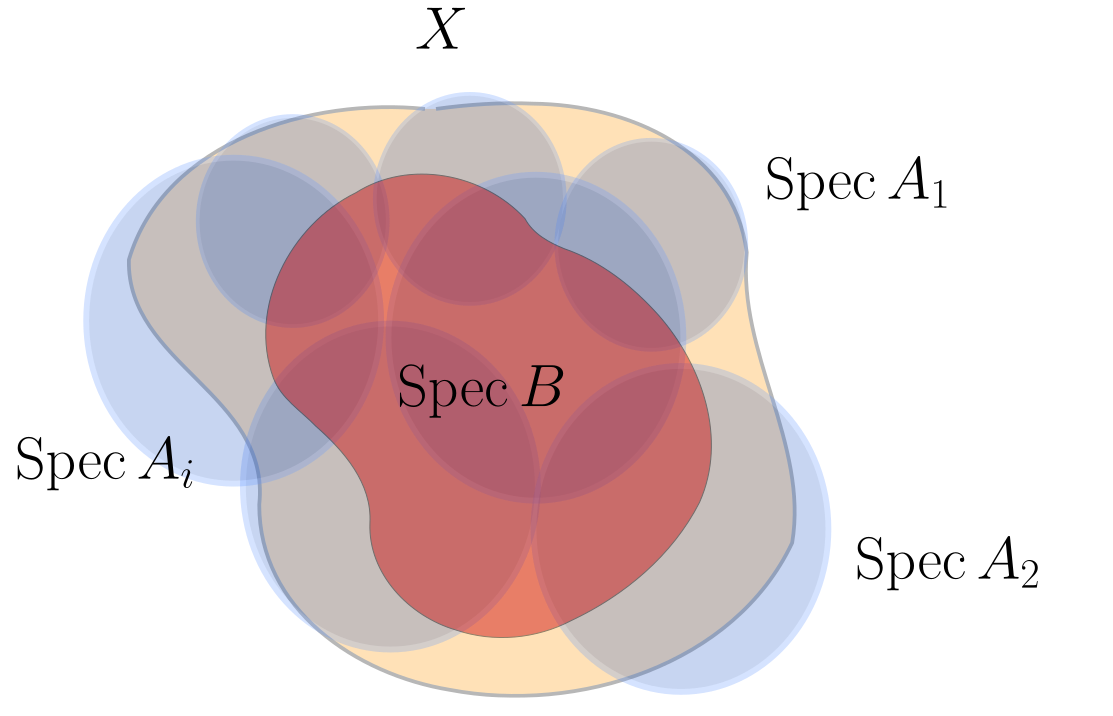Consider $$U \cap{\mathcal{U}}_i \subseteq {\mathcal{U}}_i$$ open, which can be covered by distinguished open sets. So write $$U \cap{\mathcal{U}}_i = \displaystyle\bigcup_j V_{ij}$$ with $$V_{ij} = D(f_{ij}) \subseteq \operatorname{Spec}A_{i}$$. Then $$U$$ is covered by $$\operatorname{Spec}A_i \left[ { { {f_{ij}}^{-1}} } \right]$$, i.e. spectra of local Noetherian rings. Can we conclude that $$B$$ is Noetherian from this? This will follow from the fact that we can further decompose $$V_{ij} = \displaystyle\bigcup W_{ijk}$$ where $$W_{ijk} = D_B(f_{ijk})$$.

So we want to show the following ring-theoretic statement: let $$B\in \mathsf{Ring}$$ and $$\left\{{g_i}\right\} \subseteq B$$ be a collection such that $$\operatorname{Spec}B = \displaystyle\bigcup\operatorname{Spec}B \left[ { { {g_i}^{-1}} } \right]$$ with each $$B \left[ { { {g_i}^{-1}} } \right]$$ Noetherian, then $$B$$ is necessarily Noetherian. Equivalently, we need $$\left\langle{g_i}\right\rangle = \left\langle{1}\right\rangle$$, which corresponds to $$\cap V(g_i) = \emptyset$$.

# 20 Locally Noetherian Schemes vs Noetherian Covers (Friday, October 08)

## 20.1 Proof of Theorem

Recall that we were proving the following:

$$X\in {\mathsf{Sch}}$$ is locally Noetherian iff for any affine open $$U = \operatorname{Spec}A \subseteq X$$, $$A$$ is a Noetherian ring.

Recall that we covered $$X$$ by $$U_i$$, had some affine open $$U$$ isomorphic to $$\operatorname{Spec}$$ of a ring, and then covered each intersection $$U \cap U_i$$ by distinguished opens which were $$\operatorname{Spec}R \left[ { { {f_i}^{-1}} } \right] = D(f_i) = \left\{{{\mathfrak{p}}\not\ni f_i}\right\}$$. Then $$R \left[ { { {f_i}^{-1}} } \right]$$ is Noetherian iff $$\displaystyle\bigcup_{i\in I} D(f_i) = \operatorname{Spec}R$$, which implies $$\displaystyle\bigcap_{i\in I} V(f_i) = \emptyset$$, and thus $$\not\exists p\in \operatorname{Spec}R$$ prime with $$p\ni f_i$$ for all $$i\in I$$. Then $$\left\langle{f_i {~\mathrel{\Big\vert}~}i\in I}\right\rangle = \left\langle{1}\right\rangle$$.

$$\operatorname{Spec}R$$ is quasicompact.

Let $${\mathcal{U}}\rightrightarrows\operatorname{Spec}R$$, so $$\operatorname{Spec}R = \displaystyle\bigcup_{i\in I} U_i$$, then we want to find a finite subcover. Take $$\left\{{D(f_{ij})}\right\} \rightrightarrows U_i$$; it suffices to find a finite subcover of the refined cover by distinguished opens, so reduce to $$U_i = D(f_i)$$ for each $$i$$. Using the argument from the above remark, $$\left\langle{f_i {~\mathrel{\Big\vert}~}i\in I}\right\rangle = \left\langle{1}\right\rangle$$ since this is a cover. But then there exists a finite sum $$\sum_{j=1}^N a_j f_{ij} = 1$$ for some $$a_j\in R$$, so $$\left\{{f_{ij} }\right\}_{j=1}^N = \left\langle{1}\right\rangle$$ which implies that $$\displaystyle\bigcup_{j=1}^N D(f_{ij}) = \operatorname{Spec}R$$.

Applying the proposition above, we can find a finite set $$\left\{{f_i}\right\}$$ such that $$\left\langle{f_i {~\mathrel{\Big\vert}~}i\in I}\right\rangle = \left\langle{1}\right\rangle$$ with each $$R \left[ { { {f_i}^{-1}} } \right]$$ Noetherian. We’ll use the following:

Let $$J{~\trianglelefteq~}R$$ and $$\phi_i: R\to R \left[ { { {f_i}^{-1}} } \right]$$ by the canonical localization morphism. Setting $$\left\langle{f_1,\cdots, f_s}\right\rangle = \left\langle{1}\right\rangle$$, \begin{align*} J = \cap_{i=1}^s \phi_i \qty{ \phi_i^{-1}(J) R \left[ { \scriptstyle { {f_i}^{-1}} } \right] } .\end{align*}

Note that $$\phi_i\qty{ \phi_i^{-1}(J) R \left[ { { {f}^{-1}} } \right] } \neq J$$ generally, e.g. if $$f\in J$$. So that $$J$$ is contained in the right-hand side is clear. For the reverse containment, let $$b \in \displaystyle\bigcap_i \phi_i^{-1}(\phi_i(J) R \left[ { { {f_i}^{-1}} } \right] )$$. Then $$\phi_i(b)\in \phi_i(J) R \left[ { { {f_i}^{-1}} } \right]$$ for all $$i$$, so $$b \sim a_i / f_i^{n_i}$$ in the localization for some $$a_i \in J$$.

Since $$\left\{{f_i}\right\}$$ is finite, assume that that $$n_i = n$$ for some uniform $$n$$, e.g. $$n = \max \left\{{n_i}\right\}$$. Then $$b \sim a_i/f_i^N$$, so there exist $$m_i$$ such that $$f_i^{m_i}(f_i^N b - a_i) = 0$$ in the original ring $$R$$. So now pick $$M \mathrel{\vcenter{:}}=\max\left\{{m_i}\right\}$$ to obtain $$f_i^M(f_i^N - a_i) = 0$$.

Now a trick: use that $$f_i^{M+N}b\in J$$ for all $$i$$, and the claim is that $$\left\langle{f_i^{M+N}}\right\rangle_{i\in I} = \left\langle{1}\right\rangle$$. More generally, raising all generators of a unit ideal to a fixed power still generates the unit ideal. This follows from writing $$1 = \sum_{i=1}^r c_i f_i \implies 1 = 1^M = \qty{\sum c_i f_i}^M$$, so choose $$M$$ large enough so that some $$f_i$$ occurs with an exponent of at least $$m+n$$, e.g. choosing $$M = r(m+n)$$.

If $$1 = \left\langle{x, y}\right\rangle$$, then $$\left\langle{x^2, y^2}\right\rangle = 1$$ by taking $$ax + by = 1$$ and (say) expanding $$(ax+by)^4=1$$ and noting that any term is divisible by either $$x^2$$ or $$y^2$$.

Now writing $$\sum_{i=1}^r c_i f_i^{m+n} = 1$$, we get $$\sum_{i=1}^r c_i f_i^{m+n} \in J$$, and thus $$b\in J$$.

We now know that the $$R \left[ { { {f_i}^{-1}} } \right]$$ are Noetherian. Let $$J_1 \subseteq J_2 \subseteq \cdots$$ be an ascending chain of ideals in $$R$$, we’ll show it stabilizes. Since the $$R \left[ { { {f_i}^{-1}} } \right]$$ are Noetherian, there is an ascending chain $$J_1 R \left[ { { {f_i}^{-1}} } \right] \subseteq J_2 R \left[ { { {f_i}^{-1}} } \right] \subseteq \cdots$$ in $$R \left[ { { {f_i}^{-1}} } \right]$$, which is Noetherian and thus stabilizes. So for some $$N = N(i) \gg 0$$, $$J_k R \left[ { { {f_i}^{-1}} } \right] = J_{k+1} R \left[ { { {=}^{-1}} } \right] \cdots$$ for all $$k\geq N$$. But there are only finitely many $$f_i$$, so we can choose some uniformly large $$\tilde N\gg 0$$ not depending on $$i$$ where $$J_k = J_{k+1} = \cdots$$ for all $$k\geq \tilde N$$ by applying the lemma.

On applying the lemma: use that \begin{align*} \phi_i^{-1}(J_k R \left[ { \scriptstyle { {f_i}^{-1}} } \right]) &= \phi_i^{-1}(J_{k+1}R \left[ { \scriptstyle { {f_i}^{-1}} } \right]) \quad \forall k\geq N \\ &\implies \cap_i \phi_i^{-1}(J_k R \left[ { \scriptstyle { {f_i}^{-1}} } \right]) = \cap_i \phi_i^{-1}(J_{k+1}R \left[ { \scriptstyle { {f_i}^{-1}} } \right]) \quad \forall k\geq N \\ &\implies J_{k} = J{k+1}\quad \forall k\neq N .\end{align*}

## 20.2 Other Properties

Let $$X = {\mathbb{Z}}$$ with the discrete topology (so every set is open) and set $${\mathcal{O}}_X(U) = {\mathsf{Set}}(U, k)$$ to be the local ring of arbitrary set functions. Then for $$p\in X$$, the stalks are $${\mathcal{O}}_{X, p} {\mathcal{O}}_X(\left\{{P}\right\}) = {\mathsf{Set}}(p, k) = k$$, which is local. This is a locally ringed space, since it’s locally isomorphic to $$\operatorname{Spec}R$$: we can take an open cover of such, or find a neighborhood where it holds, but $$p \in \left\{{p}\right\}$$ which is open and letting $${\mathcal{F}}\mathrel{\vcenter{:}}={ \left.{{{\mathcal{O}}_X}} \right|_{{\left\{{p}\right\}}} }$$, we have $$(\left\{{p}\right\}, {\mathcal{F}}) \cong \operatorname{Spec}k$$. Then $$X = \displaystyle\bigcup_{p\in X} \left\{{p}\right\}$$ is an open cover with no finite subcover.

So $${\mathbb{Z}}$$ with the discrete topology is not $$\operatorname{Spec}R$$ with the Zariski topology for any ring.

$$X \mathrel{\vcenter{:}}=\operatorname{Spec}\mkern 1.5mu\overline{\mkern-1.5mu{\mathbb{Q}}\mkern-1.5mu}\mkern 1.5mu[t] = \left\{{\left\langle{0}\right\rangle}\right\} \cup\left\{{\left\langle{t-a_i}\right\rangle {~\mathrel{\Big\vert}~}i\in I}\right\}$$ where $$I$$ is a countable enumeration of $$\mkern 1.5mu\overline{\mkern-1.5mu{\mathbb{Q}}\mkern-1.5mu}\mkern 1.5mu$$. Is this quasicompact?

Consider $$R \mathrel{\vcenter{:}}=\prod_{n\in {\mathbb{Z}}} {\mathbb{C}}$$, then there is a set map $$\left\{{I{~\trianglelefteq~}R}\right\} { { \, \xrightarrow{\sim}\, }}{\mathcal{P}}({\mathbb{Z}})$$, given by sending any subset to the ideal $${\mathbb{C}}\oplus {\mathbb{C}}\oplus \cdots$$ which are zeroed out at entries according to the complement of $$S$$.

What is $$\operatorname{Spec}R$$, and what is the topology? Is $$\operatorname{Spec}R$$ quasicompact?

Consider $$I \mathrel{\vcenter{:}}=\left\{{(a_i) {~\mathrel{\Big\vert}~}a_i = 0\quad i\geq N \gg 0}\right\}$$, which forms an ideal. Is $$I$$ prime? Are there prime ideals not containing $$I$$?

# 21 Monday, October 11

Last time: Noetherian isn’t a purely topological property. Today: another guiding principle in AG is that one can put properties on schemes, or alternatively on morphisms, usually to $$\operatorname{Spec}k$$.

A morphism $$X \xrightarrow{f} Y$$ of schemes is locally of finite type if there exists an affine open cover $${\mathcal{V}}\rightrightarrows Y$$ with $$V_i = \operatorname{Spec}B_i$$ such that there exists an affine open cover $${\mathcal{U}}\rightrightarrows f^{-1}({\mathcal{V}})$$ so $$U_{ij}\supseteq f^{-1}(V_i)$$ where $$U_{ij} = \operatorname{Spec}A_{ij}$$ such that the restricted function $$f: U_{ij} \to V_{i}$$ is induced by a ring morphism $$B_{i } \to A_{ij}$$ finitely generated over $$B_i$$.

This globalizes the notion of being a finitely generated ring, essentially by covering the scheme morphisms by ring morphisms with the desired property. As a special case, let $$X \in {\mathsf{Sch}}_{/ {k}}$$, so there is a morphism $$X\to \operatorname{Spec}k$$. Let $$X = \cup_j U_j$$ with $$U_j = \operatorname{Spec}A_j$$, then we want that the map $$\operatorname{Spec}A_{j}\to \operatorname{Spec}k$$ is induced by a finitely-generated ring morphism $$k\to A_j$$, so $$A_j$$ is a finitely-generated $$k{\hbox{-}}$$algebra. So this is like having a sheaf of $$k{\hbox{-}}$$algebras. As an abuse of notation/terminology, we say that $$X$$ is finite type over $$k$$ (since the target doesn’t need to be covered).

Note that a subring of a finitely generated ring need not be finitely generated!

Let $$A\in {\mathsf{Alg}_{/k} }^{{\mathrm{fg}}}$$, then $$\operatorname{Spec}A$$ is finite type over $$k$$. One can change the definition from “there exists an open cover” to “for all open covers” – this amounts to checking localizations of ring maps.

Consider $$X \mathrel{\vcenter{:}}=\mathop{\mathrm{Proj}}k[x, y] = {\mathbb{P}}^1_{/ {k}}$$, then recall that $$(D(f), { \left.{{{\mathcal{O}}_X}} \right|_{{D(f)}} }) \cong \operatorname{Spec}R \left[ { { {f}^{-1}} } \right]$$. Then \begin{align*} {\mathbb{P}}^1_{/ {k}} = D(x) {\textstyle\coprod}_f D(y) \cong {\mathbb{A}}^1_{/ {k}} {\textstyle\coprod}_f {\mathbb{A}}^1_{/ {k}} ,\end{align*} glued along inversion. Then $$k[x,y] \left[ { { {x}^{-1}} } \right] \cong k { \left[ {y\over x} \right] }$$ and $$k[x, y] \left[ { { {y}^{-1}} } \right] \cong k { \left[ {x\over y} \right] }$$. One can check that $${\mathbb{P}}^1_{/ {k}} \to \operatorname{Spec}k$$ is finite type, and this works for $${\mathbb{P}}^n$$ as well.

Note that if $$S\mathrel{\vcenter{:}}= k[x_1, \cdots, x_{n}]/I$$ for $$I$$ a homogeneous ideal, then $$\mathop{\mathrm{Proj}}S$$ is also finite type over $$k$$. We can write $$\mathop{\mathrm{Proj}}S = \displaystyle\bigcup_{i=0}^n D(\mkern 1.5mu\overline{\mkern-1.5mux\mkern-1.5mu}\mkern 1.5mu_i)$$, since taking complements yields $$\emptyset = \displaystyle\bigcap_{i=0}^n V(\mkern 1.5mu\overline{\mkern-1.5mux\mkern-1.5mu}\mkern 1.5mu_i)$$, which is the set of homogeneous prime ideals $$p\in \operatorname{Spec}S$$ with $$p\supseteq\mkern 1.5mu\overline{\mkern-1.5mux\mkern-1.5mu}\mkern 1.5mu_i$$ for all $$i$$ and $$p\not\supseteq S^+$$ the irrelevant ideal, which is empty. So $$S \left[ { { {x_i}^{-1}} } \right]$$ is a finitely generated ring, with generators of the form $$x_j/x_i$$.

$$\operatorname{Spec}k { \left[\left[ {t} \right] \right] } \to \operatorname{Spec}k$$ is not a finite type morphism, i.e. $$k{\left[\left[ t \right]\right] }$$ is not a finitely generated $$k{\hbox{-}}$$algebra. Toward a contradiction suppose $$k{\left[\left[ t \right]\right] } = \left\langle{f_1, \cdots, f_r}\right\rangle$$, so there is a ring map $$k { \left[ {f_1,\cdots, f_r} \right] } \twoheadrightarrow k{\left[\left[ t \right]\right] }$$. Take $$k= {\mathbb{Q}}$$, or any countable field, then the LHS is countable but the right-hand side is not.

Recall that a morphism $$\phi:B\to A \in \mathsf{CRing}$$ is a finite morphism if $$A$$ is finitely-generated as a $$B{\hbox{-}}$$module. A morphism $$X \xrightarrow{f} Y\in {\mathsf{Sch}}$$ is finite iff there exists an affine open cover $${\mathcal{V}}\rightrightarrows Y$$ with $$V_i = \operatorname{Spec}B_i$$ and $$f^{-1}(V_i) = \operatorname{Spec}A_i$$, and the induced ring maps $$B_i\to A_i$$ are finite.

Here the module structure is $$b\cdot a \mathrel{\vcenter{:}}=\phi(b)a$$. Note that finite type required finite generation as rings, so $$B(g_1,\cdots, g_r)\twoheadrightarrow A$$, but here we require that any $$a\in A$$ is of the form $$a = \sum_{i=1}^r \phi(b_i) a_i$$ for some $$b_i$$.

Consider $$X \mathrel{\vcenter{:}}=\operatorname{Spec}R \mathrel{\vcenter{:}}=\operatorname{Spec}{\mathbb{C}}[x, y]/ \left\langle{y^2-f(x)}\right\rangle$$ where $$f$$ has no repeated roots, which yields a hyperelliptic curve. This is a reduced ring, so $$X$$ is the scheme associated to a variety. Letting $$r_i$$ be the roots of $$f$$, we have the following: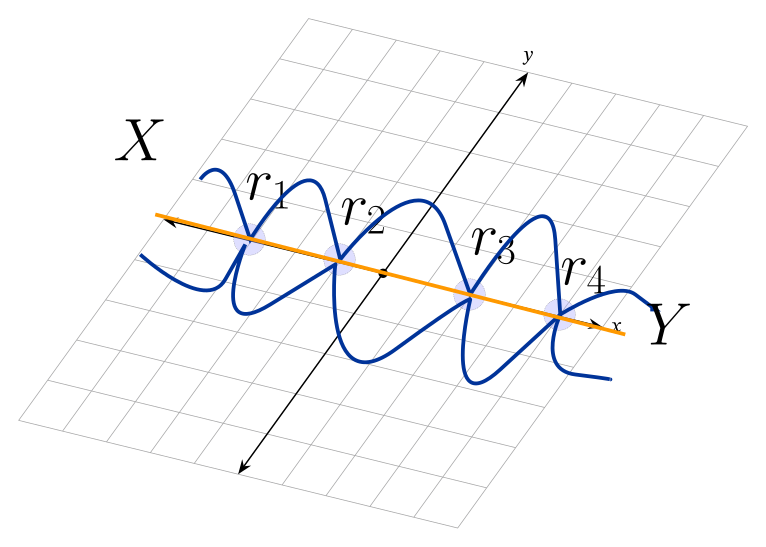Consider the function $$f:X\to Y$$ induced by the following ring map: \begin{align*} {\mathbb{C}}[x] &\to R \\ x &\mapsto x ,\end{align*} which is projection onto the axis. Note that $$R \cong {\mathbb{C}}[x] \left\langle{1}\right\rangle \oplus {\mathbb{C}}[x] \left\langle{y}\right\rangle$$ as a $${\mathbb{C}}[x]{\hbox{-}}$$module.

Consider $$\operatorname{Spec}{\mathbb{C}}[x,y,z]/\left\langle{xyz - 1}\right\rangle \to \operatorname{Spec}{\mathbb{C}}[x]$$, whose real locus yields a hyperboloid: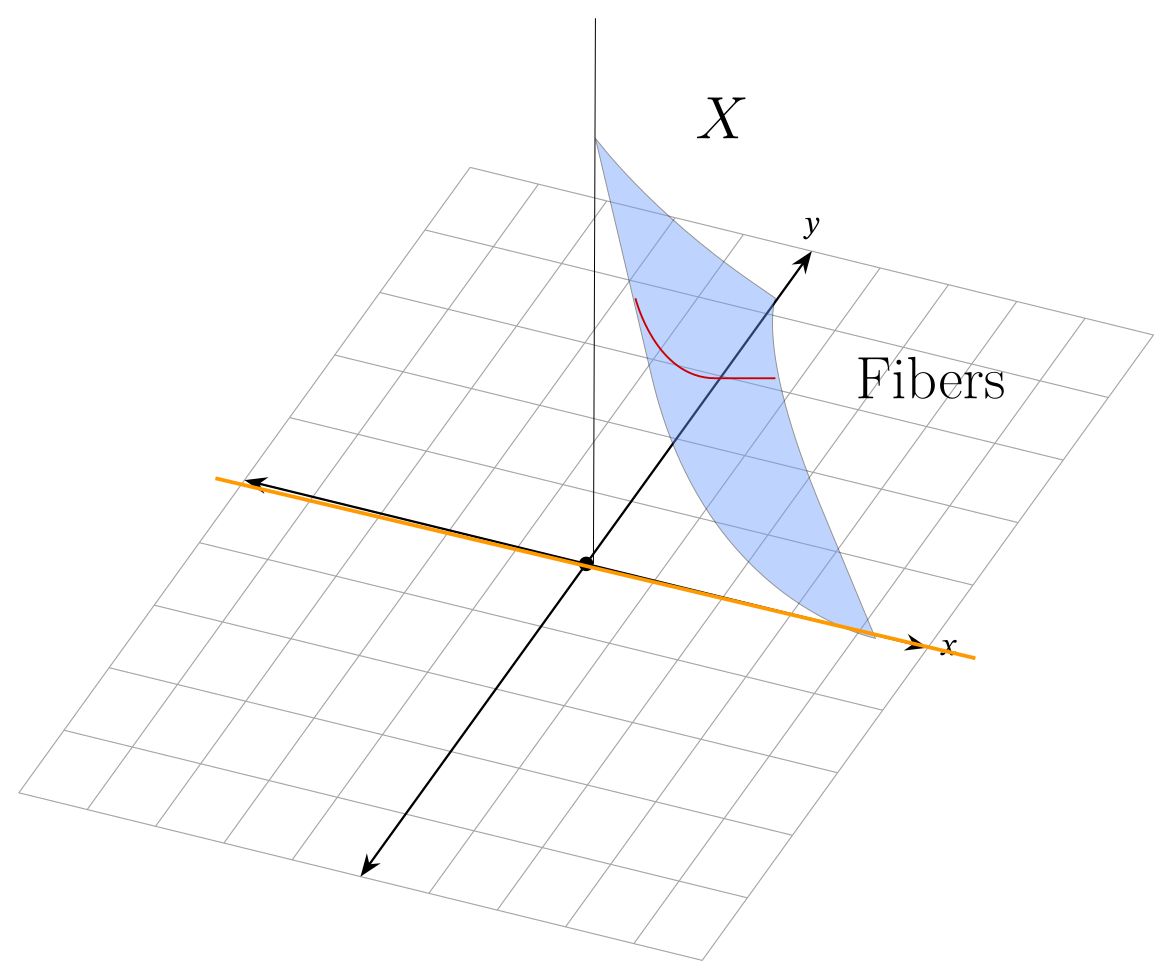Note that finite type should approximately be spec of finite type $$k{\hbox{-}}$$algebras, i.e. essentially varieties, where for finiteness the fibers should be finite.

Consider $$\operatorname{Spec}{\mathbb{C}}[x, x^{-1}] \to \operatorname{Spec}{\mathbb{C}}[x]$$, i.e. $${\mathbb{G}}_m \hookrightarrow{\mathbb{A}}^1$$. However, $${\mathbb{C}}[x, x^{-1}]$$ is not finitely-generated as a $${\mathbb{C}}[x]$$ modules, even though it has finite fibers. Given any finite set of generators, one can take $${\mathbb{C}}[x]\left\langle{f_i\over x^{k_i}}\right\rangle$$ which doesn’t contain $$1/x^{\max k_i + 1}$$.

We’ll define subschemes soon.

# 22 Wednesday, October 13

Result from last time: there doesn’t exist a surjection $$k { \left[ {f_1,\cdots, f_m} \right] } \twoheadrightarrow k { \left[ {x} \right] }$$ for any finite collection $$\left\{{f_i}\right\}_{i=1}^m$$ of polynomials. This can be checked by just considering their dimension as a $$k{\hbox{-}}$$modules, where $$\dim_k LHS = \# {\mathbb{N}}$$ and $$\dim_k RHS = \# {\mathbb{R}}$$.

We said that a morphism $$X \xrightarrow{f} Y$$ is locally finite type if it is locally modeled on $$\operatorname{Spec}A\to \operatorname{Spec}B$$ with $$B\to A$$ a finitely-generated ring morphism, and is finite if locally modeled on $$B \to A$$ module-finite. Note that in the first case, we require $$f^{-1}(U) \supseteq U\to V$$, but in the latter $$U = f^{-1}(V)$$.

As an example, the map $$D(x) \to {\mathbb{A}}^1_{/ {k}}$$ was not finite since $$k[x] \to k[x, x^{-1}]$$ is not module-finite, despite the fact that this geometrically corresponds to $${\mathbb{A}}^1\setminus\left\{{0}\right\}\hookrightarrow{\mathbb{A}}^1$$: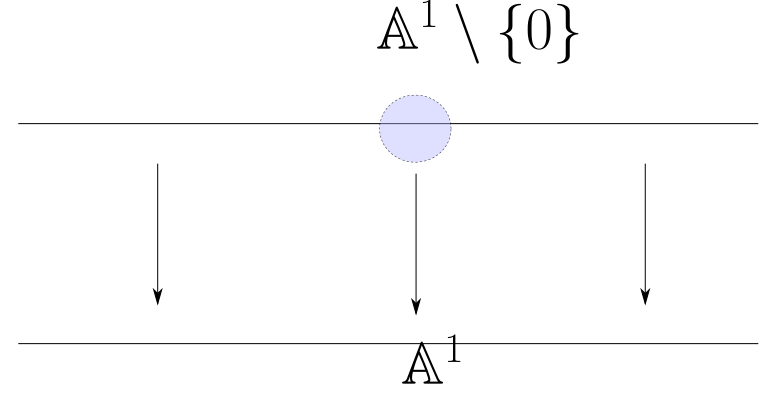Let $$p \in V$$ be a $$k{\hbox{-}}$$variety for $$k$$ an infinite field, which we can assume to be affine (so $$k[x_1, \cdots, x_{n}]/I$$ for $$I$$ reduced). Then $${\mathcal{O}}_{V, p}$$ is not not finitely-generated as a $$k{\hbox{-}}$$algebra, and $$\operatorname{Spec}{\mathcal{O}}_{V, p}\to \operatorname{Spec}k$$ is not of finite type. Consider the local ring of $${\mathbb{A}}^1_{/ {k}}$$ at the prime ideal $$p \mathrel{\vcenter{:}}=\left\langle{x}\right\rangle$$, then $$k[x] \left[ { { { \qty{ {p}^c } }^{-1}} } \right] = \left\{{f/g {~\mathrel{\Big\vert}~}g\not\in p}\right\}$$, so $$g(0) \neq 0$$ for such $$g$$. Note that this is not $$k[x] \left[ { { {x}^{-1}} } \right]$$!

Idea: there are only finitely many denominators: if \begin{align*} \phi: k { \left[ {f_1/g_1, \cdots, f_r/g_r} \right] } \twoheadrightarrow k[x] \left[ { \scriptstyle { { \qty{ {p}^c } }^{-1}} } \right] ,\end{align*} then $$\operatorname{im}\phi$$ contains contains those $$f/g$$ such that $$V(g) \subseteq \cup V(g_i)$$, so such a $$\phi$$ can not exist. Note that this is still true for $$k$$ a finite field, just not by this proof.

## 22.1 Open/Closed Subschemes

Given $$X\in {\mathsf{Sch}}$$, an open subscheme of $$X$$ is an open subset $$U \subseteq { {\left\lvert {X} \right\rvert} }$$ with structure sheaf $${\mathcal{O}}_U = { \left.{{{\mathcal{O}}_X}} \right|_{{U}} }$$.

Why does $$(U, {\mathcal{O}}_U) \in \mathsf{Loc}\mathsf{RingSp}$$ form a scheme? One needs to check that it’s locally isomorphic to the spectrum of a ring: let $$\left\{{X_i}\right\}\rightrightarrows X$$ be an open affine cover, then $$U_i\mathrel{\vcenter{:}}= U \cap X_i$$ is open in $$U$$ and in $$X_i$$, so can be covered by distinguished opens $$V_{ij}$$. But then $$\left\{{V_{ij}}\right\}\rightrightarrows U$$ is a cover by affine schemes. The inclusion $$(U, {\mathcal{O}}_U) \hookrightarrow(X, {\mathcal{O}}_X)$$ is clearly a morphism in $$\mathsf{Loc}\mathsf{RingSp}$$.

The inclusion above is an open immersion.

A small dictionary

AG Rest of Math
Immersion Embedding
Nothing! Immersion

Here I write $${ {\left\lvert {X} \right\rvert} } \mathrel{\vcenter{:}}=\mathrm{sp}(X)$$ as an alternative for Hartshorne’s notation.

A closed immersion is a morphism $$X \xrightarrow{f} Y\in {\mathsf{Sch}}$$ such that

1. The induced map $${ {\left\lvert {X} \right\rvert} }\to { {\left\lvert {Y} \right\rvert} } \in {\mathsf{Top}}$$ is a homeomorphism onto a closed subset of $${ {\left\lvert {Y} \right\rvert} }$$,
2. $$f^\#: {\mathcal{O}}_Y \twoheadrightarrow f_* {\mathcal{O}}_X \in {\mathsf{Sh}}(Y)$$ is surjective.

Set $$U = D(f) \subseteq \operatorname{Spec}A$$ defines an open immersion $$\operatorname{Spec}A \left[ { { {f}^{-1}} } \right] \to \operatorname{Spec}A$$. So this corresponds to the ring map $$A\hookrightarrow A \left[ { { {f}^{-1}} } \right]$$ since $$\operatorname{Spec}A \left[ { { {f}^{-1}} } \right] \subseteq \operatorname{Spec}A$$ are those ideals not containing $$f$$.

Consider $$U \mathrel{\vcenter{:}}={\mathbb{A}}^2_{/ {k}} \setminus\left\{{{\left[ {x, y} \right]}}\right\} \hookrightarrow{\mathbb{A}}^2_{/ {k}}$$. Then $$\left\{{{\left[ {x, y} \right]}}\right\} = V(x, y)$$, and this is a subscheme of an affine scheme which is not itself affine. One can use that $$\dim D(f)^c \geq 1$$

Prove that this is not affine. Hint: check $${\mathcal{O}}_U = k[x,y]$$,1 and use that for any $$X \in {\mathsf{Aff}}{\mathsf{Sch}}$$ we have $${\mathcal{O}}_X(X) = R$$. However, $$\operatorname{Spec}k[x, y] = {\mathbb{A}}^2\neq U$$.

Check “affinization?” It fills in holes of at codimension at most 2, and satisfies a universal property. Consider $$X = {\mathbb{A}}^1\times {\mathbb{P}}^1$$.

All examples are locally of this form: $$F:X\hookrightarrow Y = \operatorname{Spec}A$$ where $${ {\left\lvert {X} \right\rvert} }\to U \subseteq { {\left\lvert {Y} \right\rvert} }$$ maps homeomorphically onto a closed subset. Recall that the closed subsets are of the form $$U = V(I)$$, and here we need $$f^\#: {\mathcal{O}}_Y \to f_* {\mathcal{O}}_X$$ surjective. Let $$X = \operatorname{Spec}A/I$$, and recall that every surjective ring map is of the form $$A\to A/I$$. Here $$q: A\twoheadrightarrow A/I$$ where $${\mathfrak{p}}\mapsfrom q^{-1}({\mathfrak{p}})$$, so we get some map $$\operatorname{Spec}A/I\to \operatorname{Spec}A$$, and this is homeomorphism onto $$V(I) \subseteq \operatorname{Spec}A$$: \begin{align*} \operatorname{Spec}A/I &\to V(I) \\ {\mathfrak{p}}&\mapsto q^{-1}({\mathfrak{p}}) \supseteq I \\ q({\mathfrak{q}}) &\mapsfrom {\mathfrak{q}} .\end{align*} We also get an induced map $$A \left[ { { {g}^{-1}} } \right] \to (A/I) \left[ { { {g}^{-1}} } \right]$$, which is precisely \begin{align*} f^\#(D(g)): {\mathcal{O}}_Y(D(g)) \to {\mathcal{O}}_X(f^{-1}(D(g))) \end{align*} and is thus surjective. Since it’s surjective on a basis, by gluing it’ll be surjective on the entire space.